Скачать презентацию Data Mining Concepts and Techniques Chapter 6

19a15b7a68bd2d66ef343b2b4bfdf9bb.ppt

• Количество слайдов: 78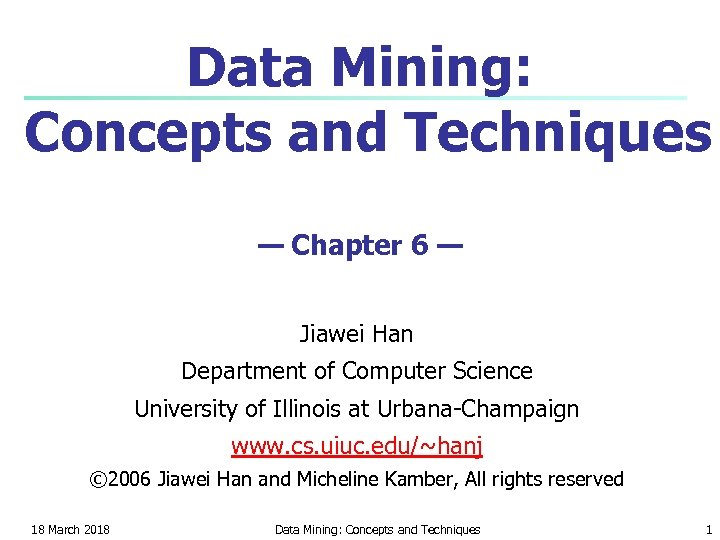Data Mining: Concepts and Techniques — Chapter 6 — Jiawei Han Department of Computer Science University of Illinois at Urbana-Champaign www. cs. uiuc. edu/~hanj © 2006 Jiawei Han and Micheline Kamber, All rights reserved 18 March 2018 Data Mining: Concepts and Techniques 118 March 2018 Data Mining: Concepts and Techniques 2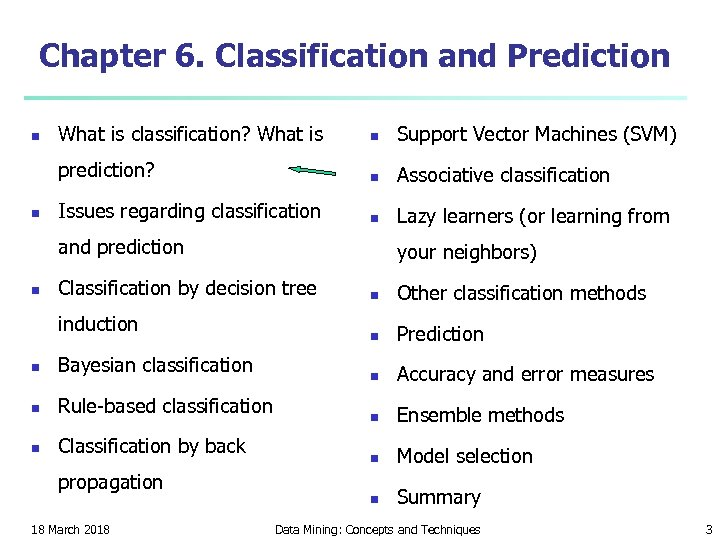Chapter 6. Classification and Prediction n What is classification? What is n Support Vector Machines (SVM) prediction? n n Associative classification Issues regarding classification n Lazy learners (or learning from and prediction n your neighbors) Classification by decision tree induction n Bayesian classification n Rule-based classification n Classification by back propagation 18 March 2018 n Other classification methods n Prediction n Accuracy and error measures n Ensemble methods n Model selection n Summary Data Mining: Concepts and Techniques 3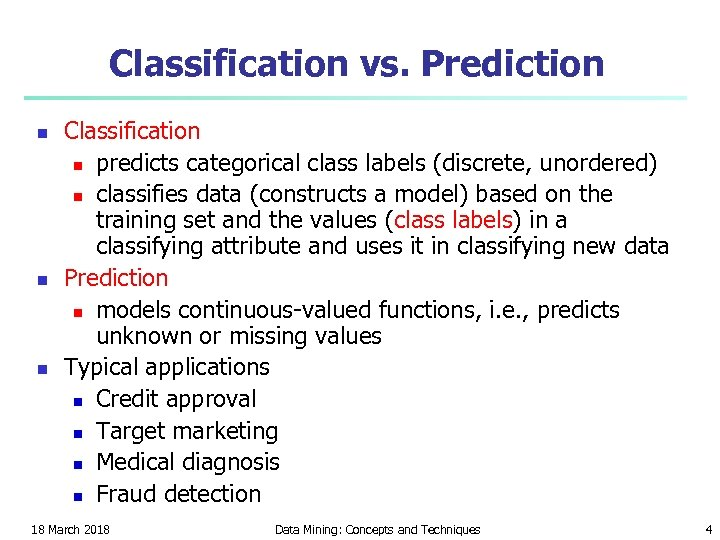Classification vs. Prediction n Classification n predicts categorical class labels (discrete, unordered) n classifies data (constructs a model) based on the training set and the values (class labels) in a classifying attribute and uses it in classifying new data Prediction n models continuous-valued functions, i. e. , predicts unknown or missing values Typical applications n Credit approval n Target marketing n Medical diagnosis n Fraud detection 18 March 2018 Data Mining: Concepts and Techniques 4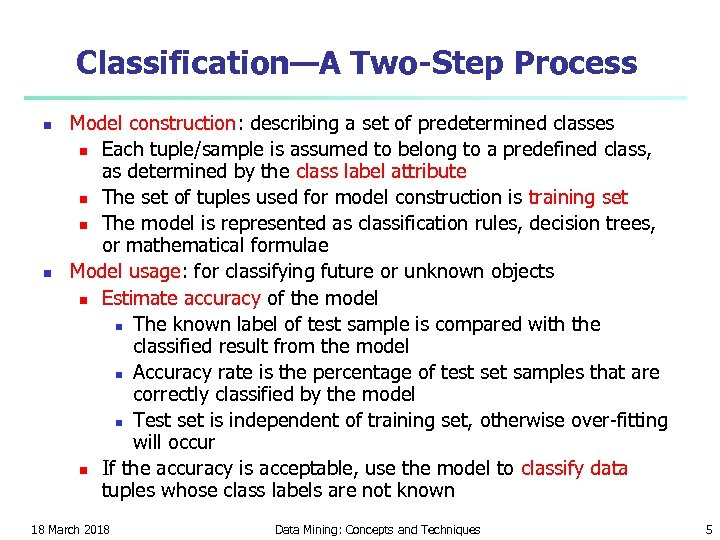Classification—A Two-Step Process n n Model construction: describing a set of predetermined classes n Each tuple/sample is assumed to belong to a predefined class, as determined by the class label attribute n The set of tuples used for model construction is training set n The model is represented as classification rules, decision trees, or mathematical formulae Model usage: for classifying future or unknown objects n Estimate accuracy of the model n The known label of test sample is compared with the classified result from the model n Accuracy rate is the percentage of test set samples that are correctly classified by the model n Test set is independent of training set, otherwise over-fitting will occur n If the accuracy is acceptable, use the model to classify data tuples whose class labels are not known 18 March 2018 Data Mining: Concepts and Techniques 5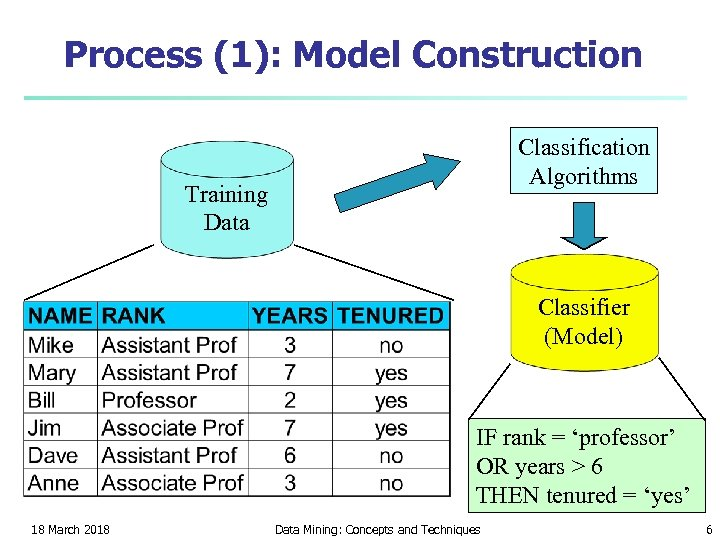Process (1): Model Construction Classification Algorithms Training Data Classifier (Model) IF rank = ‘professor’ OR years > 6 THEN tenured = ‘yes’ 18 March 2018 Data Mining: Concepts and Techniques 6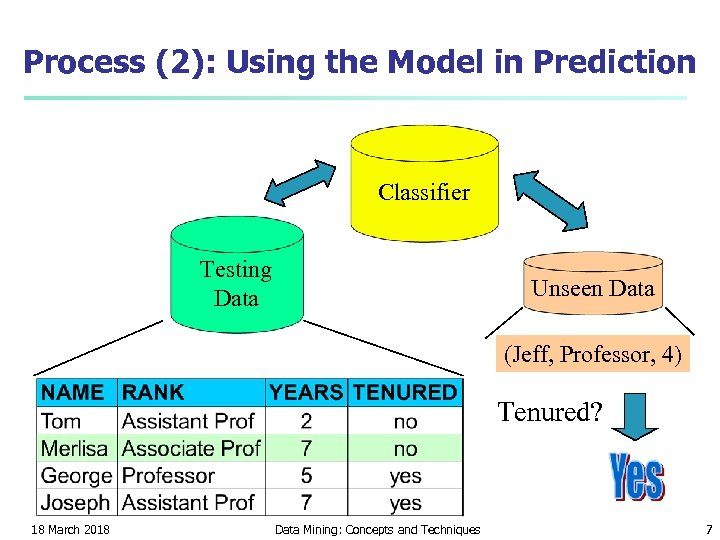Process (2): Using the Model in Prediction Classifier Testing Data Unseen Data (Jeff, Professor, 4) Tenured? 18 March 2018 Data Mining: Concepts and Techniques 7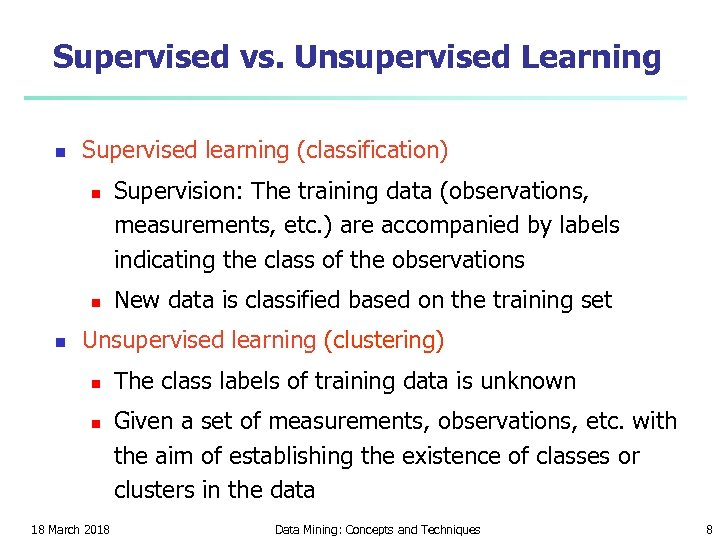Supervised vs. Unsupervised Learning n Supervised learning (classification) n n n Supervision: The training data (observations, measurements, etc. ) are accompanied by labels indicating the class of the observations New data is classified based on the training set Unsupervised learning (clustering) n n 18 March 2018 The class labels of training data is unknown Given a set of measurements, observations, etc. with the aim of establishing the existence of classes or clusters in the data Data Mining: Concepts and Techniques 8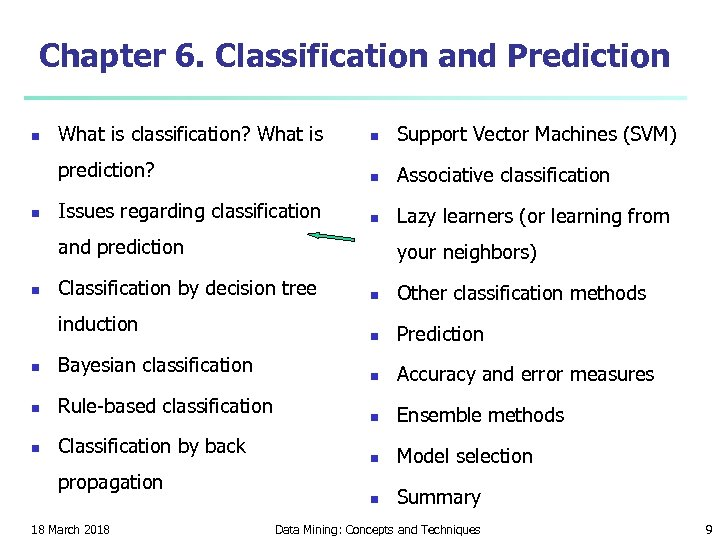Chapter 6. Classification and Prediction n What is classification? What is n Support Vector Machines (SVM) prediction? n n Associative classification Issues regarding classification n Lazy learners (or learning from and prediction n your neighbors) Classification by decision tree induction n Bayesian classification n Rule-based classification n Classification by back propagation 18 March 2018 n Other classification methods n Prediction n Accuracy and error measures n Ensemble methods n Model selection n Summary Data Mining: Concepts and Techniques 9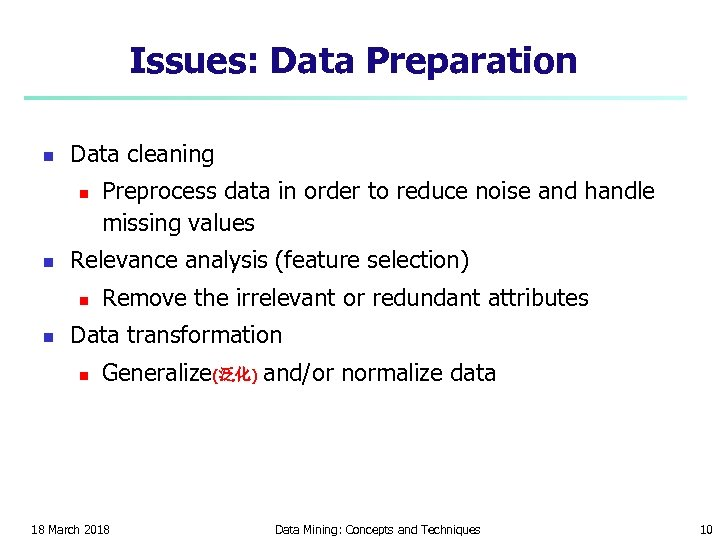Issues: Data Preparation n Data cleaning n n Relevance analysis (feature selection) n n Preprocess data in order to reduce noise and handle missing values Remove the irrelevant or redundant attributes Data transformation n Generalize(泛化) and/or normalize data 18 March 2018 Data Mining: Concepts and Techniques 10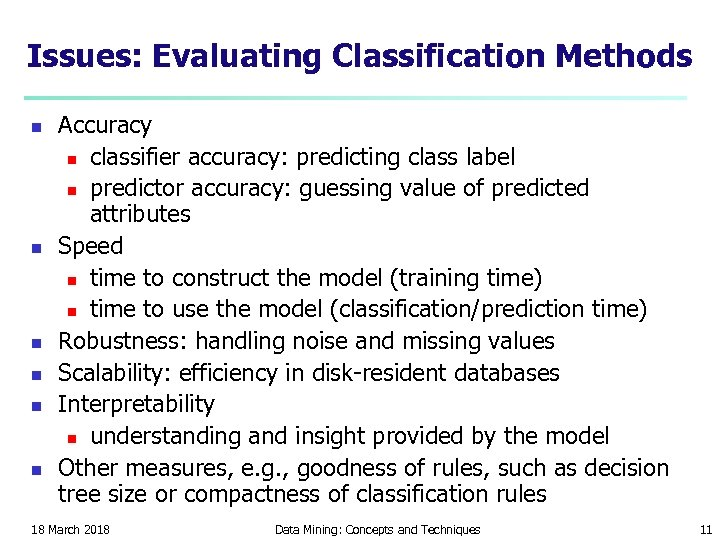Issues: Evaluating Classification Methods n n n Accuracy n classifier accuracy: predicting class label n predictor accuracy: guessing value of predicted attributes Speed n time to construct the model (training time) n time to use the model (classification/prediction time) Robustness: handling noise and missing values Scalability: efficiency in disk-resident databases Interpretability n understanding and insight provided by the model Other measures, e. g. , goodness of rules, such as decision tree size or compactness of classification rules 18 March 2018 Data Mining: Concepts and Techniques 11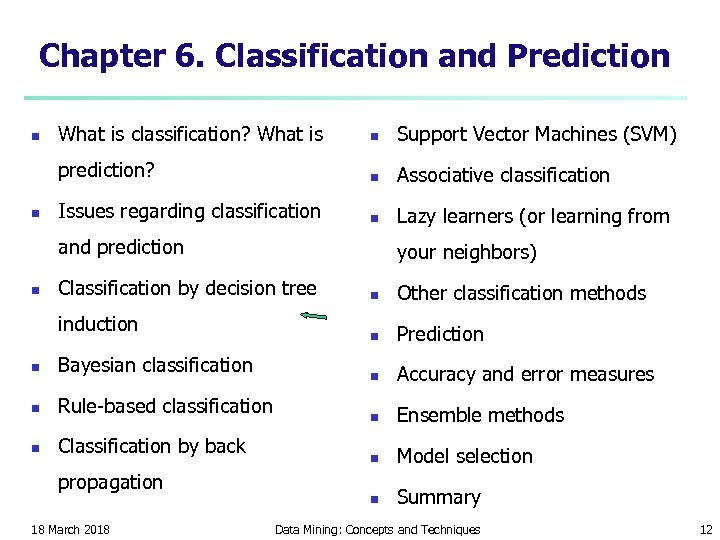Chapter 6. Classification and Prediction n What is classification? What is n Support Vector Machines (SVM) prediction? n n Associative classification Issues regarding classification n Lazy learners (or learning from and prediction n your neighbors) Classification by decision tree induction n Bayesian classification n Rule-based classification n Classification by back propagation 18 March 2018 n Other classification methods n Prediction n Accuracy and error measures n Ensemble methods n Model selection n Summary Data Mining: Concepts and Techniques 12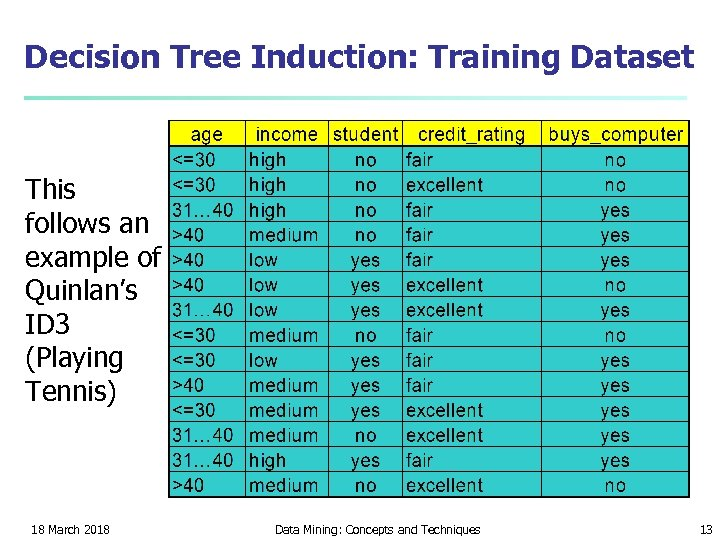Decision Tree Induction: Training Dataset This follows an example of Quinlan’s ID 3 (Playing Tennis) 18 March 2018 Data Mining: Concepts and Techniques 13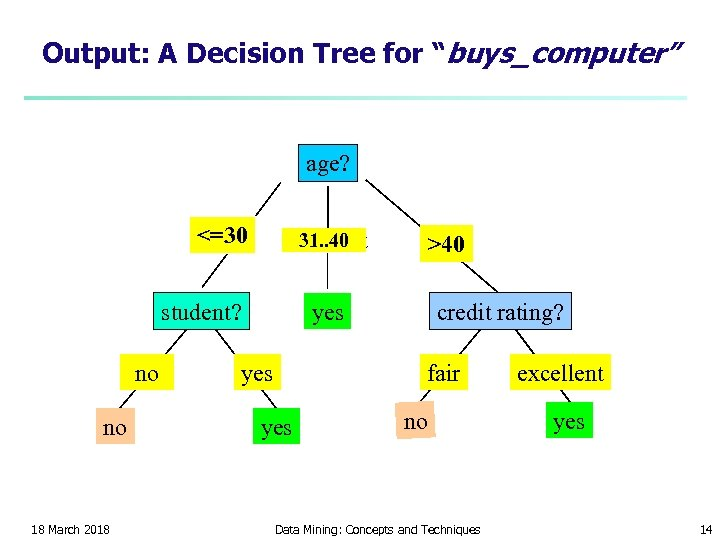Output: A Decision Tree for “buys_computer” age? <=30 31. . 40 overcast student? no no 18 March 2018 >40 credit rating? yes fair yes no Data Mining: Concepts and Techniques excellent yes 14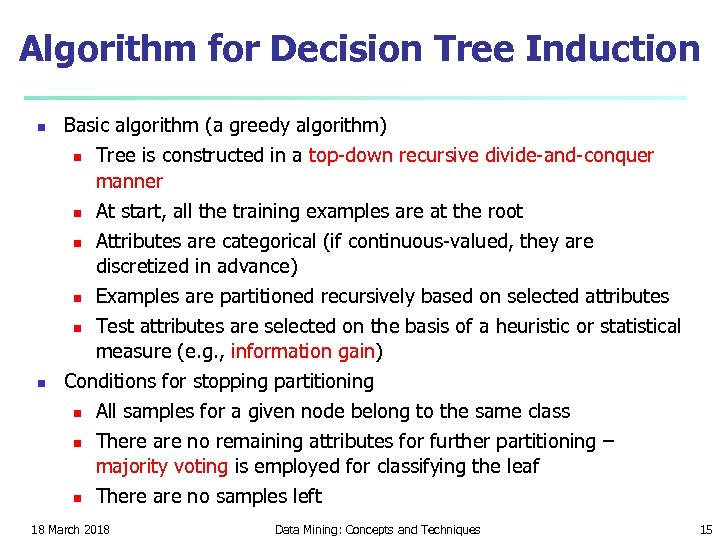Algorithm for Decision Tree Induction n n Basic algorithm (a greedy algorithm) n Tree is constructed in a top-down recursive divide-and-conquer manner n At start, all the training examples are at the root n Attributes are categorical (if continuous-valued, they are discretized in advance) n Examples are partitioned recursively based on selected attributes n Test attributes are selected on the basis of a heuristic or statistical measure (e. g. , information gain) Conditions for stopping partitioning n All samples for a given node belong to the same class n There are no remaining attributes for further partitioning – majority voting is employed for classifying the leaf n There are no samples left 18 March 2018 Data Mining: Concepts and Techniques 15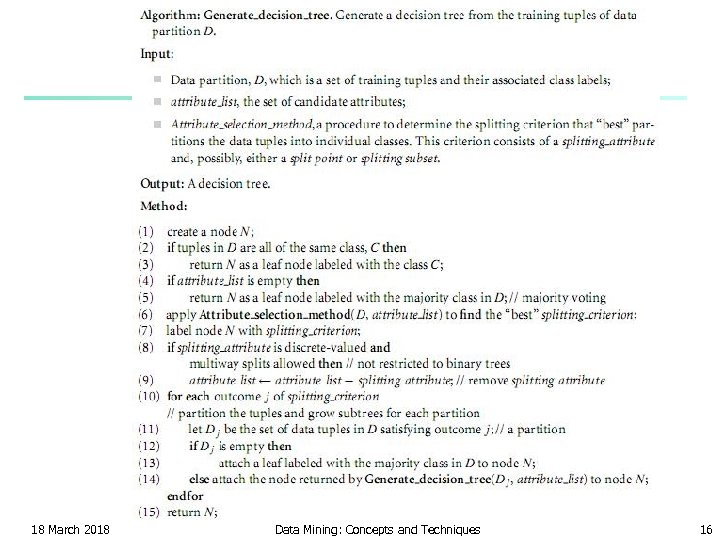18 March 2018 Data Mining: Concepts and Techniques 16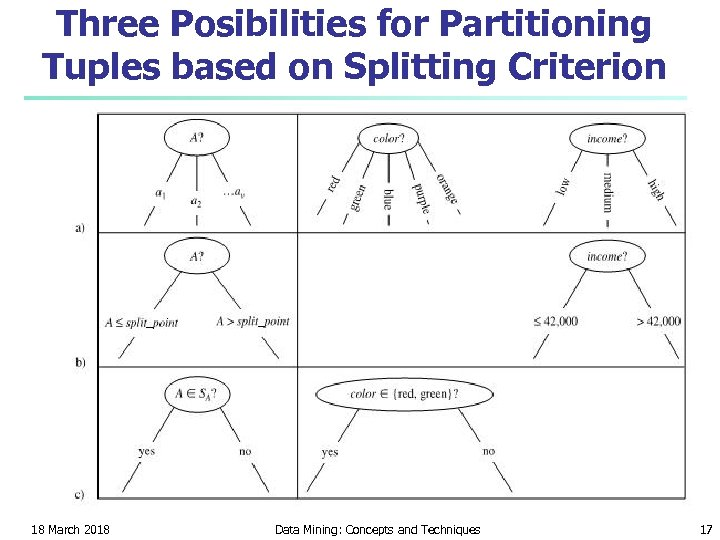Three Posibilities for Partitioning Tuples based on Splitting Criterion 18 March 2018 Data Mining: Concepts and Techniques 17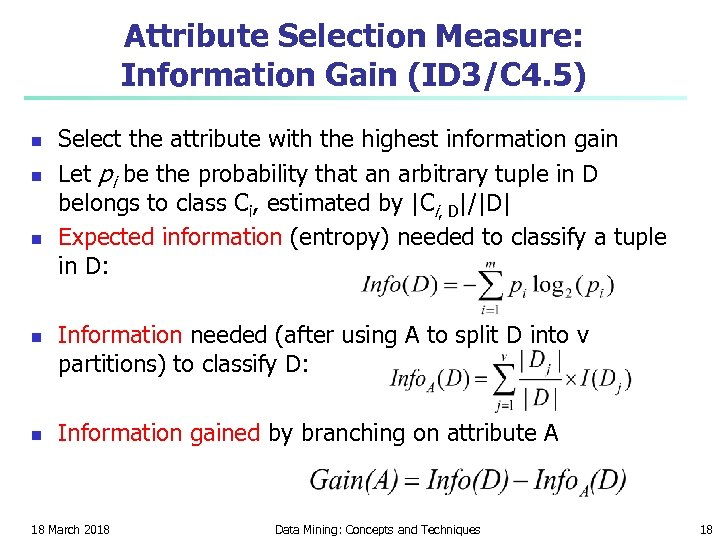Attribute Selection Measure: Information Gain (ID 3/C 4. 5) n n n Select the attribute with the highest information gain Let pi be the probability that an arbitrary tuple in D belongs to class Ci, estimated by |Ci, D|/|D| Expected information (entropy) needed to classify a tuple in D: Information needed (after using A to split D into v partitions) to classify D: Information gained by branching on attribute A 18 March 2018 Data Mining: Concepts and Techniques 18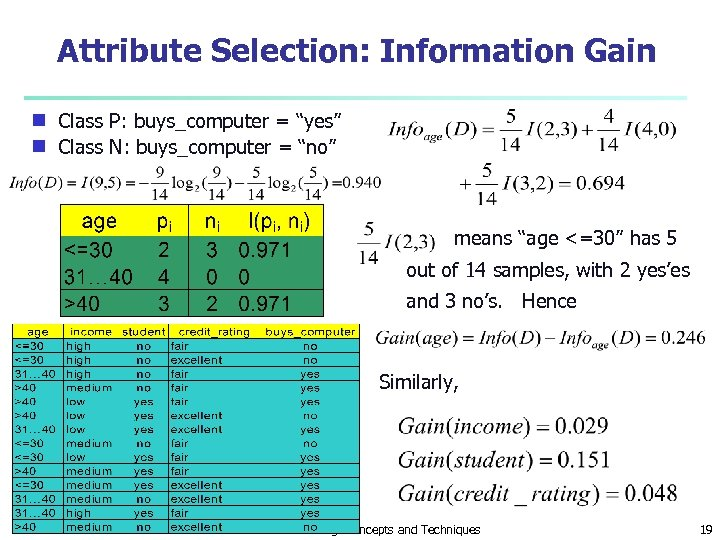Attribute Selection: Information Gain Class P: buys_computer = “yes” g Class N: buys_computer = “no” g means “age <=30” has 5 out of 14 samples, with 2 yes’es and 3 no’s. Hence Similarly, 18 March 2018 Data Mining: Concepts and Techniques 19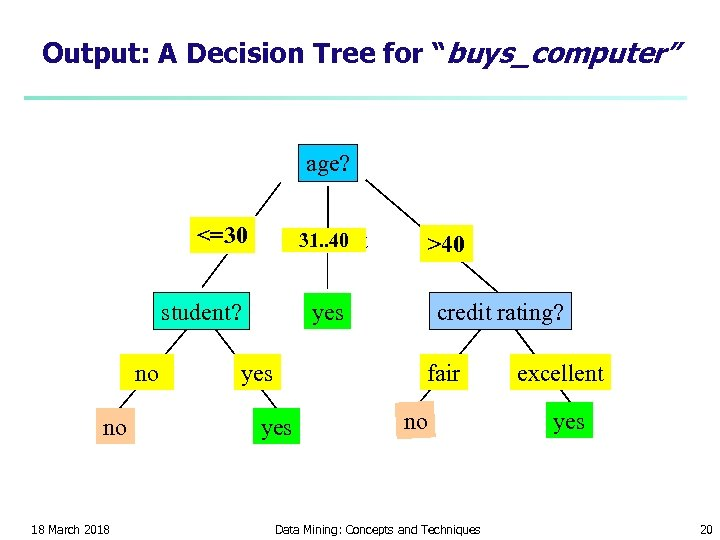Output: A Decision Tree for “buys_computer” age? <=30 31. . 40 overcast student? no no 18 March 2018 >40 credit rating? yes fair yes no Data Mining: Concepts and Techniques excellent yes 20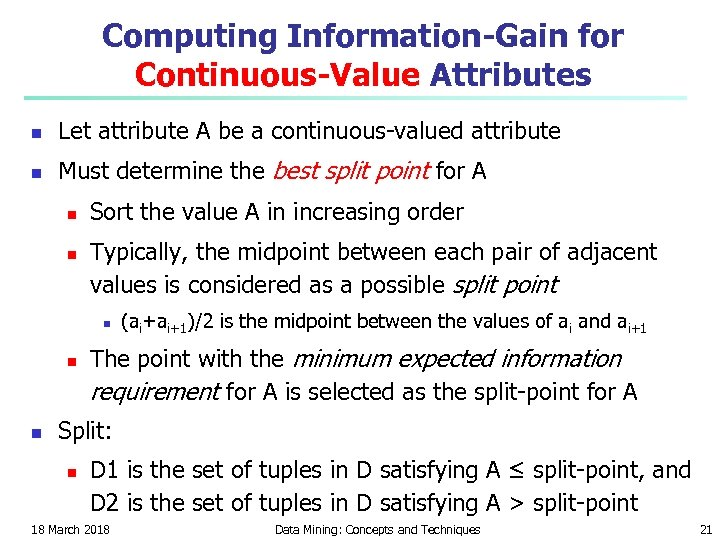Computing Information-Gain for Continuous-Value Attributes n Let attribute A be a continuous-valued attribute n Must determine the best split point for A n n Sort the value A in increasing order Typically, the midpoint between each pair of adjacent values is considered as a possible split point n n n (ai+ai+1)/2 is the midpoint between the values of ai and ai+1 The point with the minimum expected information requirement for A is selected as the split-point for A Split: n D 1 is the set of tuples in D satisfying A ≤ split-point, and D 2 is the set of tuples in D satisfying A > split-point 18 March 2018 Data Mining: Concepts and Techniques 21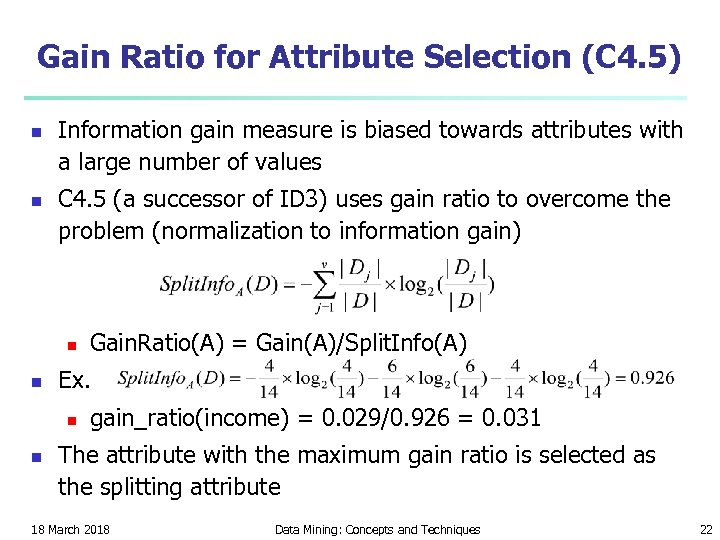Gain Ratio for Attribute Selection (C 4. 5) n n Information gain measure is biased towards attributes with a large number of values C 4. 5 (a successor of ID 3) uses gain ratio to overcome the problem (normalization to information gain) n n Ex. n n Gain. Ratio(A) = Gain(A)/Split. Info(A) gain_ratio(income) = 0. 029/0. 926 = 0. 031 The attribute with the maximum gain ratio is selected as the splitting attribute 18 March 2018 Data Mining: Concepts and Techniques 22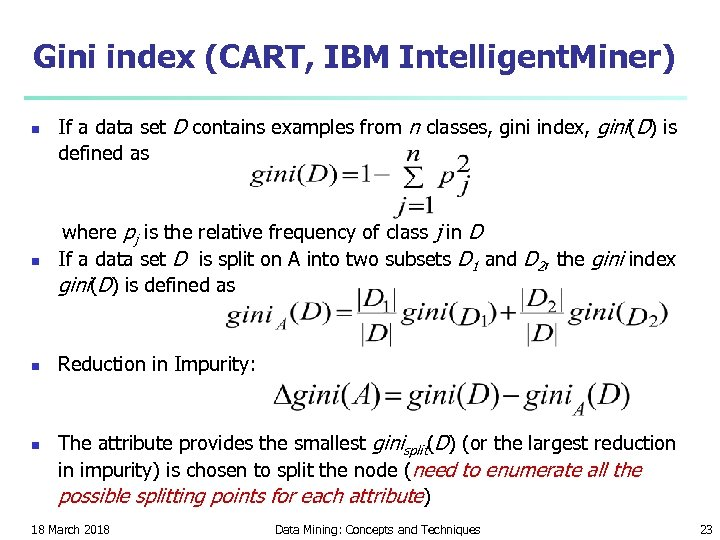Gini index (CART, IBM Intelligent. Miner) n If a data set D contains examples from n classes, gini index, gini(D) is defined as n where pj is the relative frequency of class j in D If a data set D is split on A into two subsets D 1 and D 2, the gini index gini(D) is defined as n Reduction in Impurity: n The attribute provides the smallest ginisplit(D) (or the largest reduction in impurity) is chosen to split the node (need to enumerate all the possible splitting points for each attribute) 18 March 2018 Data Mining: Concepts and Techniques 23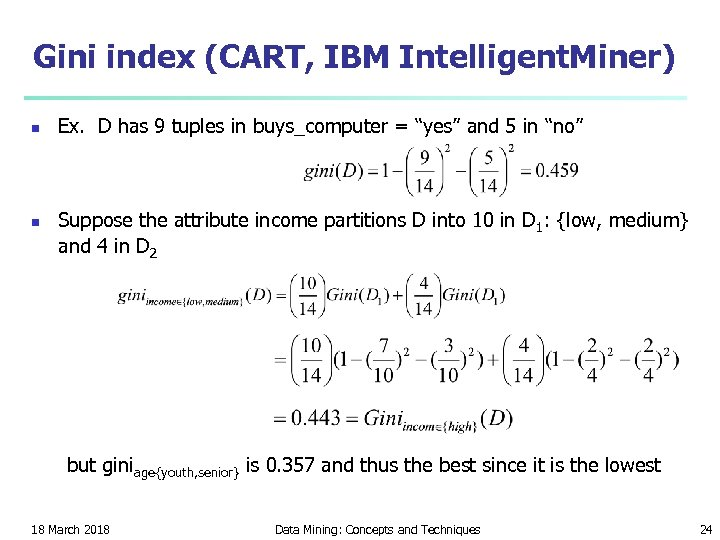Gini index (CART, IBM Intelligent. Miner) n n Ex. D has 9 tuples in buys_computer = “yes” and 5 in “no” Suppose the attribute income partitions D into 10 in D 1: {low, medium} and 4 in D 2 but giniage{youth, senior} is 0. 357 and thus the best since it is the lowest 18 March 2018 Data Mining: Concepts and Techniques 24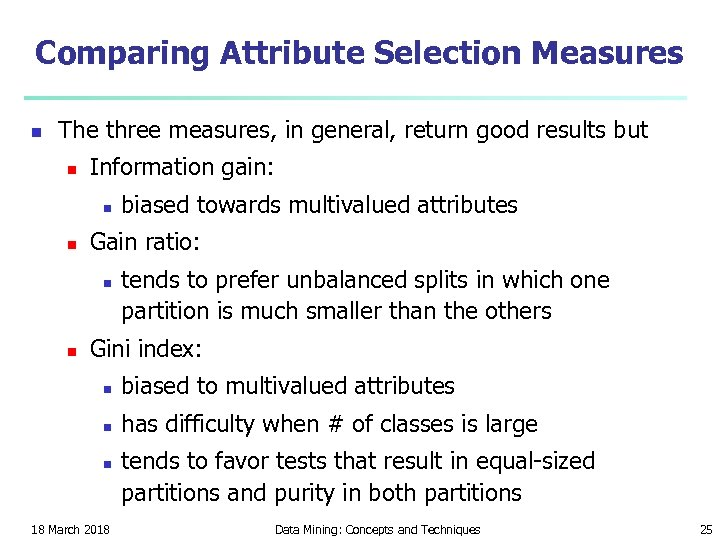Comparing Attribute Selection Measures n The three measures, in general, return good results but n Information gain: n n Gain ratio: n n biased towards multivalued attributes tends to prefer unbalanced splits in which one partition is much smaller than the others Gini index: n biased to multivalued attributes n has difficulty when # of classes is large n 18 March 2018 tends to favor tests that result in equal-sized partitions and purity in both partitions Data Mining: Concepts and Techniques 25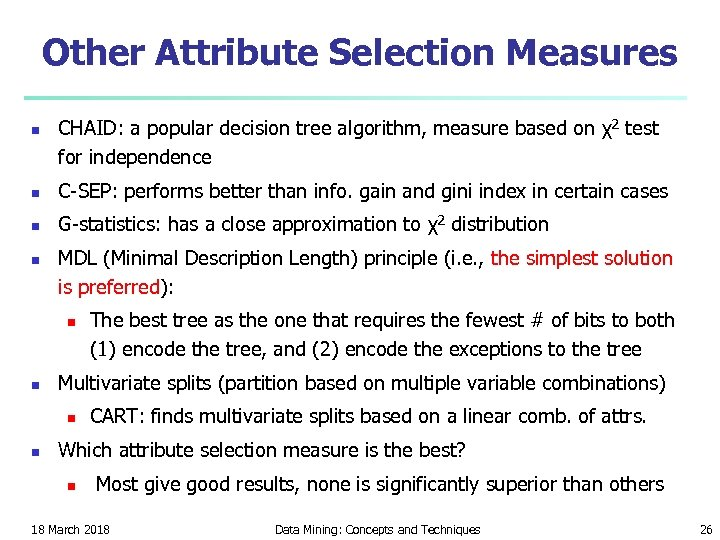Other Attribute Selection Measures n CHAID: a popular decision tree algorithm, measure based on χ2 test for independence n C-SEP: performs better than info. gain and gini index in certain cases n G-statistics: has a close approximation to χ2 distribution n MDL (Minimal Description Length) principle (i. e. , the simplest solution is preferred): n n Multivariate splits (partition based on multiple variable combinations) n n The best tree as the one that requires the fewest # of bits to both (1) encode the tree, and (2) encode the exceptions to the tree CART: finds multivariate splits based on a linear comb. of attrs. Which attribute selection measure is the best? n Most give good results, none is significantly superior than others 18 March 2018 Data Mining: Concepts and Techniques 26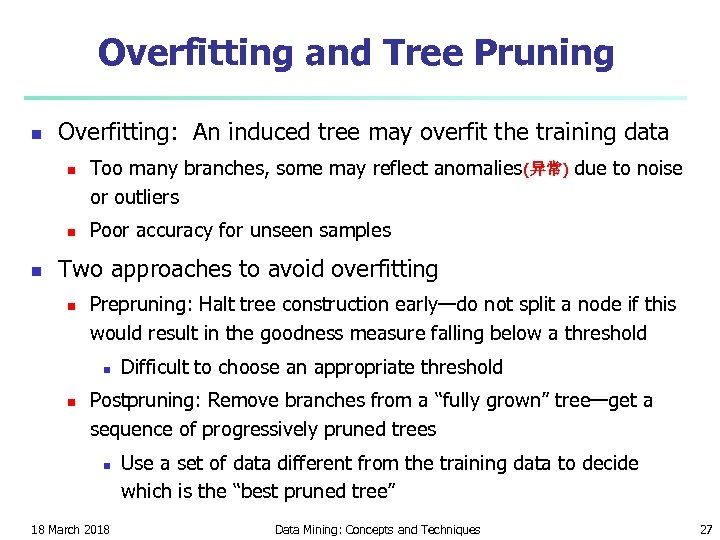Overfitting and Tree Pruning n Overfitting: An induced tree may overfit the training data n n n Too many branches, some may reflect anomalies(异常) due to noise or outliers Poor accuracy for unseen samples Two approaches to avoid overfitting n Prepruning: Halt tree construction early—do not split a node if this would result in the goodness measure falling below a threshold n n Difficult to choose an appropriate threshold Postpruning: Remove branches from a “fully grown” tree—get a sequence of progressively pruned trees n 18 March 2018 Use a set of data different from the training data to decide which is the “best pruned tree” Data Mining: Concepts and Techniques 27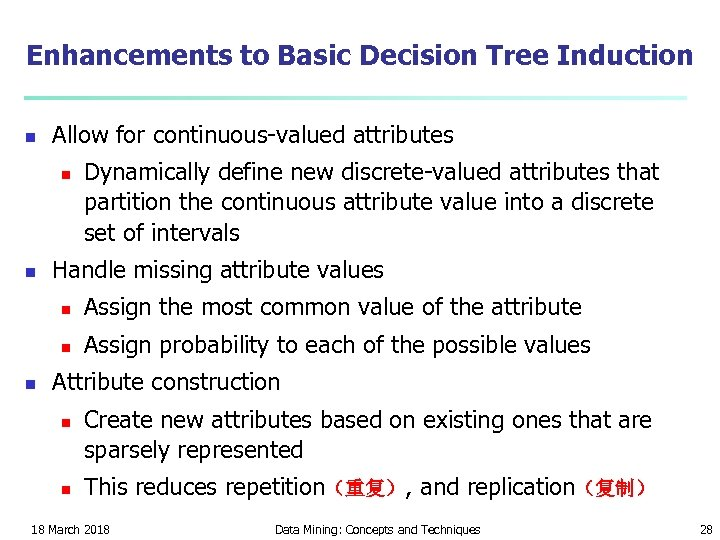Enhancements to Basic Decision Tree Induction n Allow for continuous-valued attributes n n Dynamically define new discrete-valued attributes that partition the continuous attribute value into a discrete set of intervals Handle missing attribute values n n n Assign the most common value of the attribute Assign probability to each of the possible values Attribute construction n n Create new attributes based on existing ones that are sparsely represented This reduces repetition（重复）, and replication（复制） 18 March 2018 Data Mining: Concepts and Techniques 28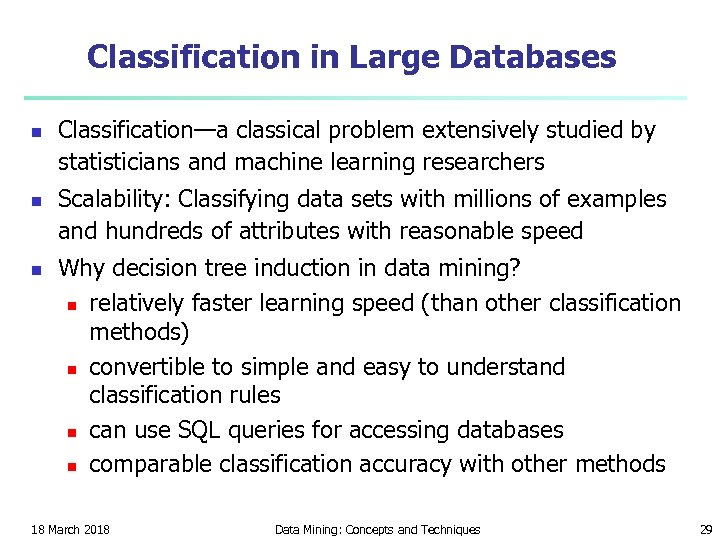Classification in Large Databases n n n Classification—a classical problem extensively studied by statisticians and machine learning researchers Scalability: Classifying data sets with millions of examples and hundreds of attributes with reasonable speed Why decision tree induction in data mining? n relatively faster learning speed (than other classification methods) n convertible to simple and easy to understand classification rules n can use SQL queries for accessing databases n comparable classification accuracy with other methods 18 March 2018 Data Mining: Concepts and Techniques 29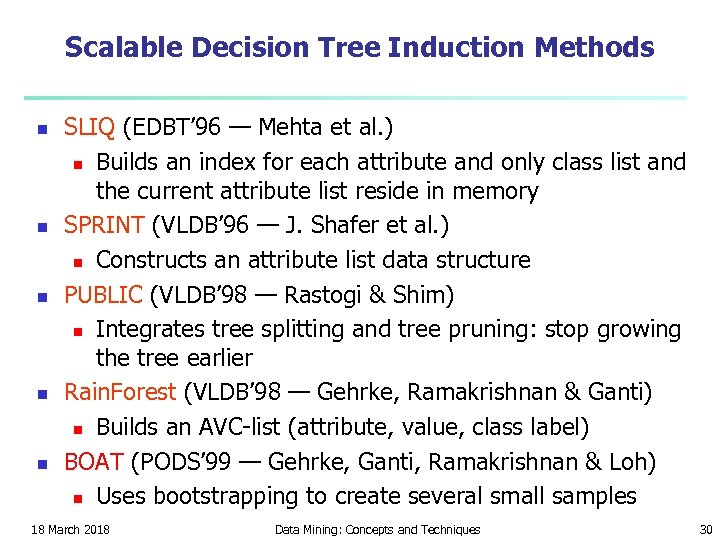Scalable Decision Tree Induction Methods n n n SLIQ (EDBT’ 96 — Mehta et al. ) n Builds an index for each attribute and only class list and the current attribute list reside in memory SPRINT (VLDB’ 96 — J. Shafer et al. ) n Constructs an attribute list data structure PUBLIC (VLDB’ 98 — Rastogi & Shim) n Integrates tree splitting and tree pruning: stop growing the tree earlier Rain. Forest (VLDB’ 98 — Gehrke, Ramakrishnan & Ganti) n Builds an AVC-list (attribute, value, class label) BOAT (PODS’ 99 — Gehrke, Ganti, Ramakrishnan & Loh) n Uses bootstrapping to create several small samples 18 March 2018 Data Mining: Concepts and Techniques 30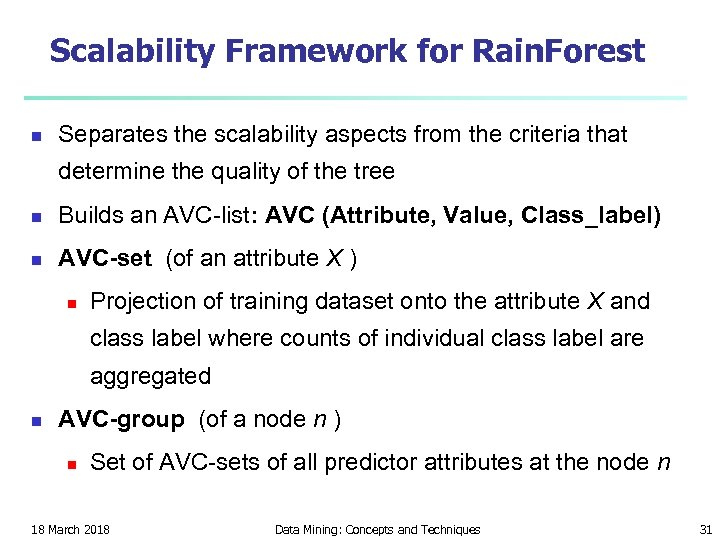Scalability Framework for Rain. Forest n Separates the scalability aspects from the criteria that determine the quality of the tree n Builds an AVC-list: AVC (Attribute, Value, Class_label) n AVC-set (of an attribute X ) n Projection of training dataset onto the attribute X and class label where counts of individual class label are aggregated n AVC-group (of a node n ) n Set of AVC-sets of all predictor attributes at the node n 18 March 2018 Data Mining: Concepts and Techniques 31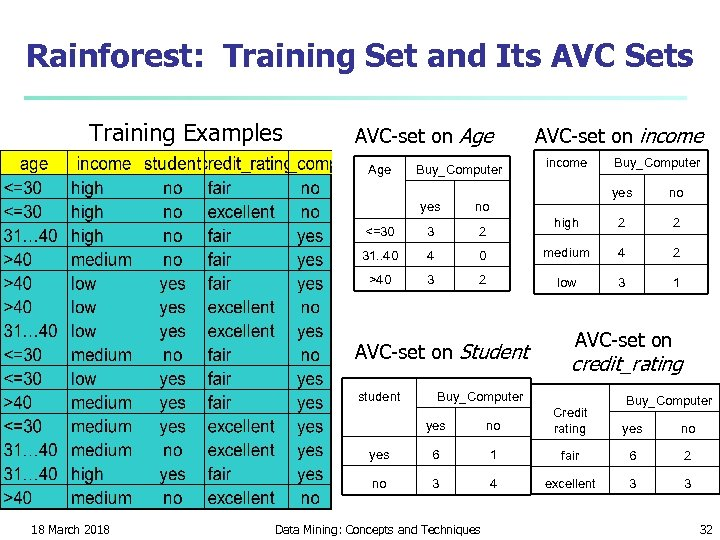Rainforest: Training Set and Its AVC Sets Training Examples AVC-set on Age Buy_Computer AVC-set on income yes no high 2 2 0 medium 4 2 2 low 3 1 yes no <=30 3 2 31. . 40 4 >40 3 AVC-set on Student student Buy_Computer AVC-set on credit_rating Buy_Computer yes 6 1 fair 6 2 no 18 March 2018 no Credit rating 3 4 excellent 3 3 Data Mining: Concepts and Techniques yes no 32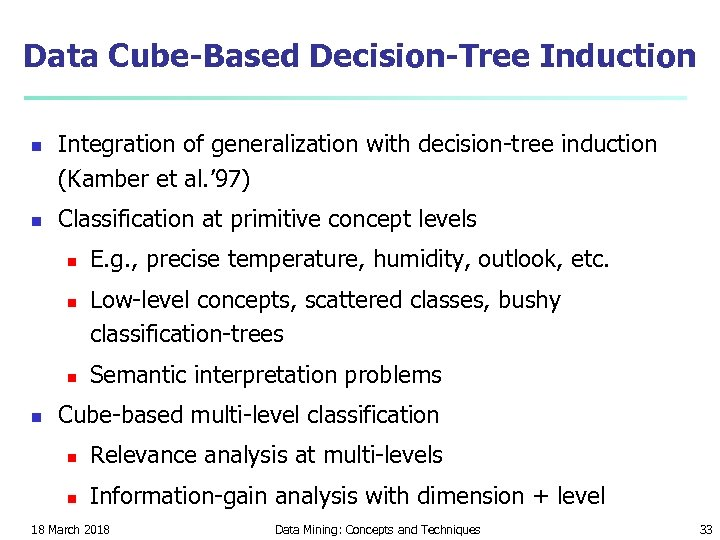Data Cube-Based Decision-Tree Induction n n Integration of generalization with decision-tree induction (Kamber et al. ’ 97) Classification at primitive concept levels n n E. g. , precise temperature, humidity, outlook, etc. Low-level concepts, scattered classes, bushy classification-trees Semantic interpretation problems Cube-based multi-level classification n Relevance analysis at multi-levels n Information-gain analysis with dimension + level 18 March 2018 Data Mining: Concepts and Techniques 33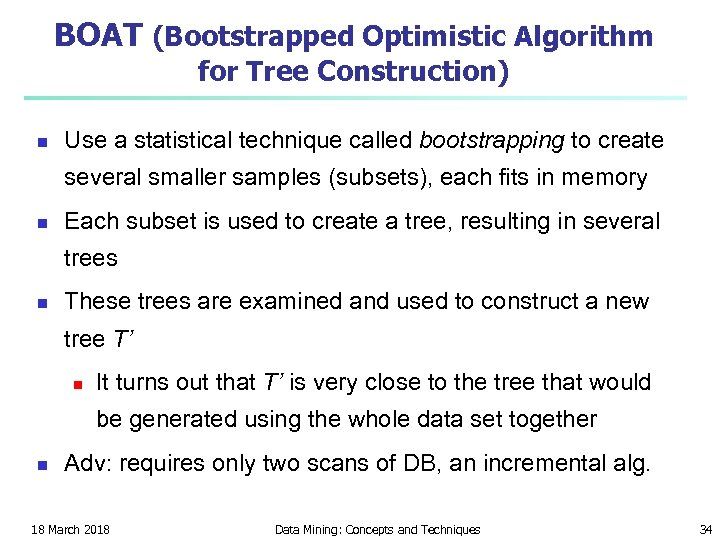BOAT (Bootstrapped Optimistic Algorithm for Tree Construction) n Use a statistical technique called bootstrapping to create several smaller samples (subsets), each fits in memory n Each subset is used to create a tree, resulting in several trees n These trees are examined and used to construct a new tree T’ n It turns out that T’ is very close to the tree that would be generated using the whole data set together n Adv: requires only two scans of DB, an incremental alg. 18 March 2018 Data Mining: Concepts and Techniques 34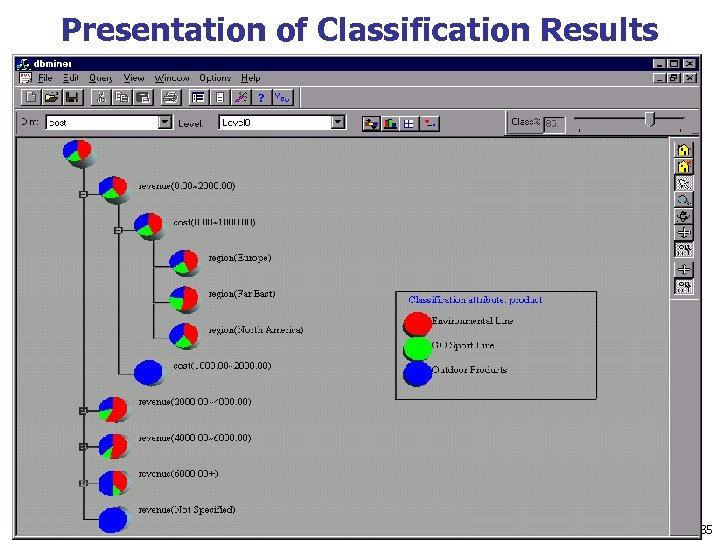Presentation of Classification Results 18 March 2018 Data Mining: Concepts and Techniques 35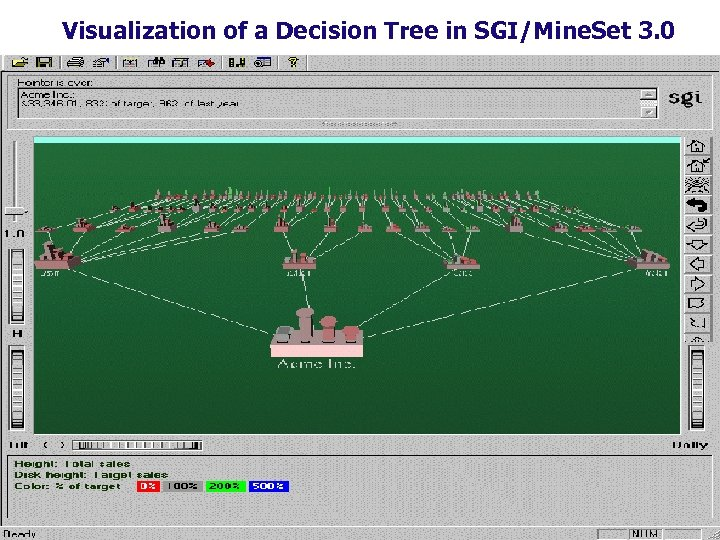Visualization of a Decision Tree in SGI/Mine. Set 3. 0 18 March 2018 Data Mining: Concepts and Techniques 36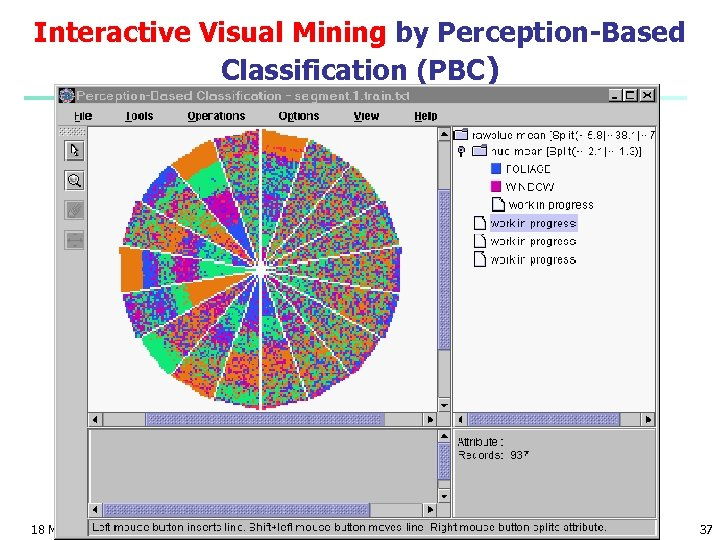Interactive Visual Mining by Perception-Based Classification (PBC) 18 March 2018 Data Mining: Concepts and Techniques 37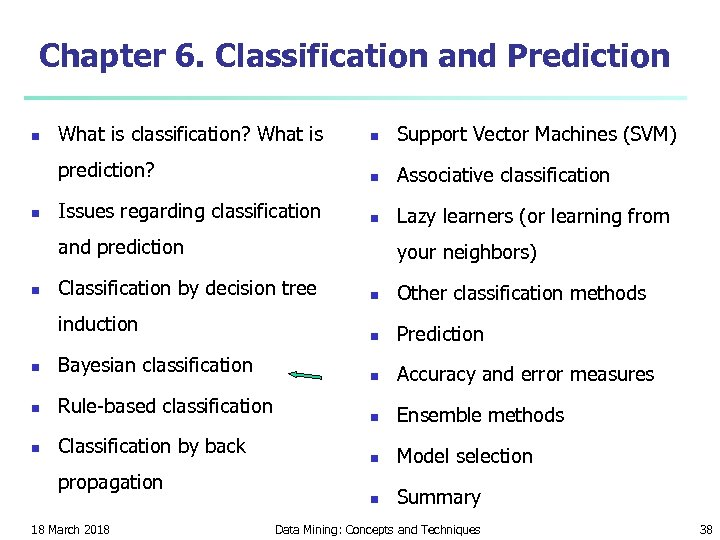Chapter 6. Classification and Prediction n What is classification? What is n Support Vector Machines (SVM) prediction? n n Associative classification Issues regarding classification n Lazy learners (or learning from and prediction n your neighbors) Classification by decision tree induction n Bayesian classification n Rule-based classification n Classification by back propagation 18 March 2018 n Other classification methods n Prediction n Accuracy and error measures n Ensemble methods n Model selection n Summary Data Mining: Concepts and Techniques 38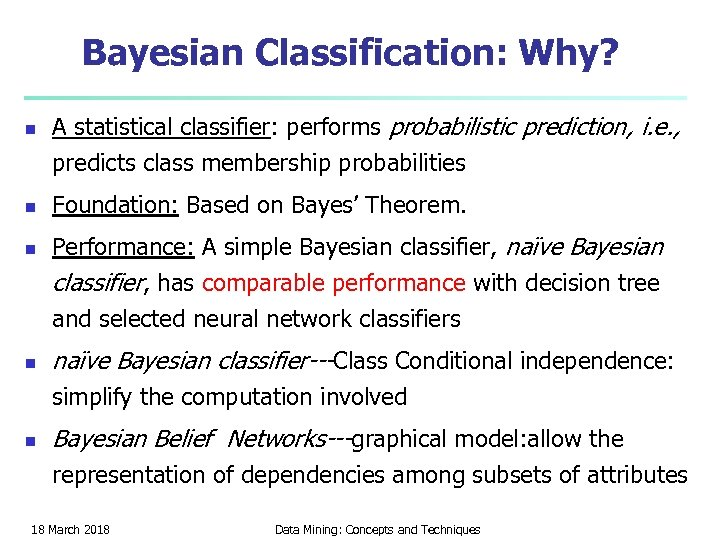Bayesian Classification: Why? n n A statistical classifier: performs probabilistic prediction, i. e. , predicts class membership probabilities Foundation: Based on Bayes’ Theorem. Performance: A simple Bayesian classifier, naïve Bayesian classifier, has comparable performance with decision tree and selected neural network classifiers naïve Bayesian classifier---Class Conditional independence: simplify the computation involved n Bayesian Belief Networks---graphical model: allow the representation of dependencies among subsets of attributes 18 March 2018 Data Mining: Concepts and Techniques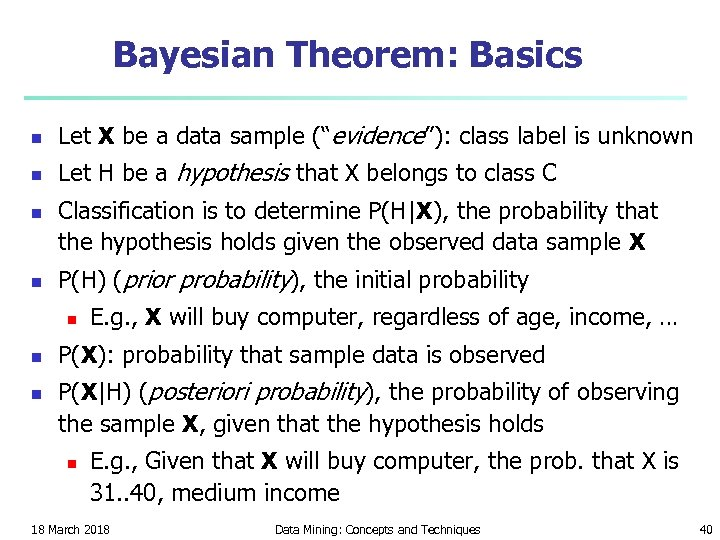Bayesian Theorem: Basics n Let X be a data sample (“evidence”): class label is unknown n Let H be a hypothesis that X belongs to class C n n Classification is to determine P(H|X), the probability that the hypothesis holds given the observed data sample X P(H) (prior probability), the initial probability n n n E. g. , X will buy computer, regardless of age, income, … P(X): probability that sample data is observed P(X|H) (posteriori probability), the probability of observing the sample X, given that the hypothesis holds n E. g. , Given that X will buy computer, the prob. that X is 31. . 40, medium income 18 March 2018 Data Mining: Concepts and Techniques 40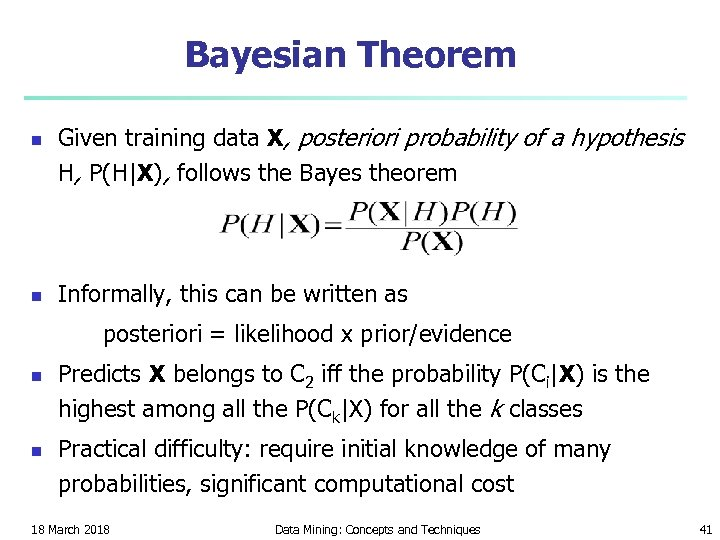Bayesian Theorem n n Given training data X, posteriori probability of a hypothesis H, P(H|X), follows the Bayes theorem Informally, this can be written as posteriori = likelihood x prior/evidence n n Predicts X belongs to C 2 iff the probability P(Ci|X) is the highest among all the P(Ck|X) for all the k classes Practical difficulty: require initial knowledge of many probabilities, significant computational cost 18 March 2018 Data Mining: Concepts and Techniques 41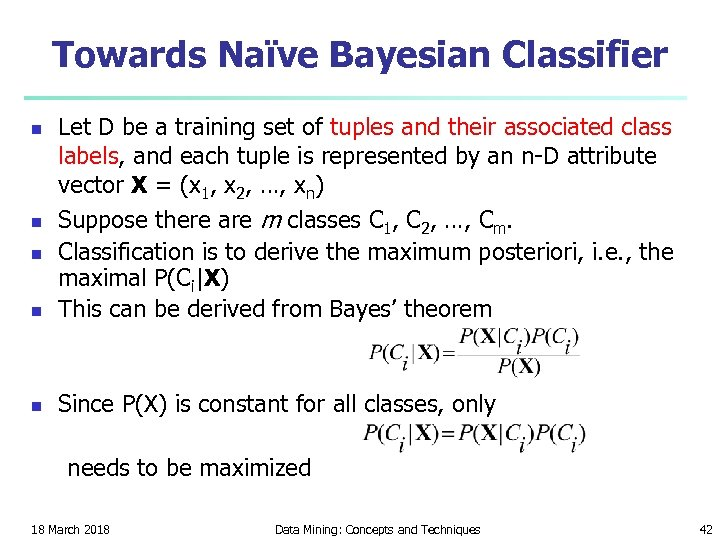Towards Naïve Bayesian Classifier n Let D be a training set of tuples and their associated class labels, and each tuple is represented by an n-D attribute vector X = (x 1, x 2, …, xn) Suppose there are m classes C 1, C 2, …, Cm. Classification is to derive the maximum posteriori, i. e. , the maximal P(Ci|X) This can be derived from Bayes’ theorem n Since P(X) is constant for all classes, only n needs to be maximized 18 March 2018 Data Mining: Concepts and Techniques 42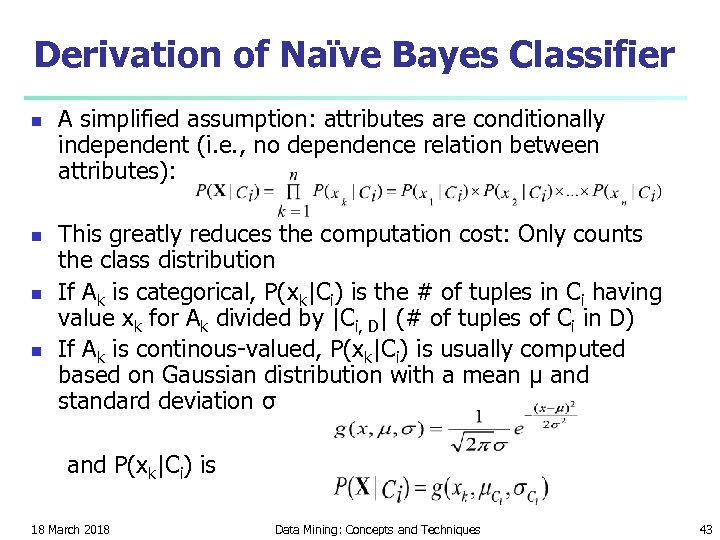Derivation of Naïve Bayes Classifier n n A simplified assumption: attributes are conditionally independent (i. e. , no dependence relation between attributes): This greatly reduces the computation cost: Only counts the class distribution If Ak is categorical, P(xk|Ci) is the # of tuples in Ci having value xk for Ak divided by |Ci, D| (# of tuples of Ci in D) If Ak is continous-valued, P(xk|Ci) is usually computed based on Gaussian distribution with a mean μ and standard deviation σ and P(xk|Ci) is 18 March 2018 Data Mining: Concepts and Techniques 43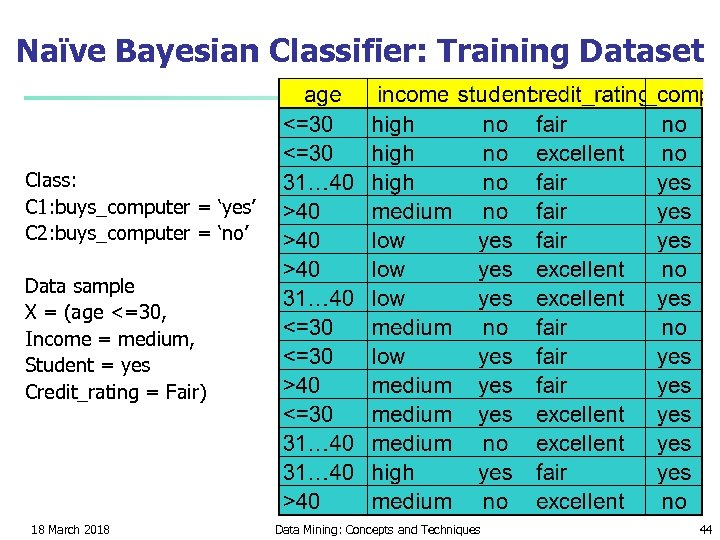Naïve Bayesian Classifier: Training Dataset Class: C 1: buys_computer = ‘yes’ C 2: buys_computer = ‘no’ Data sample X = (age <=30, Income = medium, Student = yes Credit_rating = Fair) 18 March 2018 Data Mining: Concepts and Techniques 44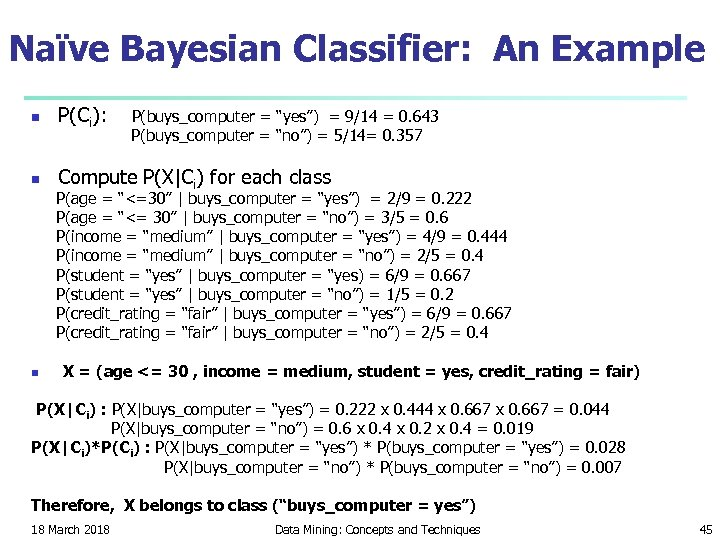Naïve Bayesian Classifier: An Example n P(Ci): n Compute P(X|Ci) for each class P(buys_computer = “yes”) = 9/14 = 0. 643 P(buys_computer = “no”) = 5/14= 0. 357 P(age = “<=30” | buys_computer = “yes”) = 2/9 = 0. 222 P(age = “<= 30” | buys_computer = “no”) = 3/5 = 0. 6 P(income = “medium” | buys_computer = “yes”) = 4/9 = 0. 444 P(income = “medium” | buys_computer = “no”) = 2/5 = 0. 4 P(student = “yes” | buys_computer = “yes) = 6/9 = 0. 667 P(student = “yes” | buys_computer = “no”) = 1/5 = 0. 2 P(credit_rating = “fair” | buys_computer = “yes”) = 6/9 = 0. 667 P(credit_rating = “fair” | buys_computer = “no”) = 2/5 = 0. 4 n X = (age <= 30 , income = medium, student = yes, credit_rating = fair) P(X|Ci) : P(X|buys_computer = “yes”) = 0. 222 x 0. 444 x 0. 667 = 0. 044 P(X|buys_computer = “no”) = 0. 6 x 0. 4 x 0. 2 x 0. 4 = 0. 019 P(X|Ci)*P(Ci) : P(X|buys_computer = “yes”) * P(buys_computer = “yes”) = 0. 028 P(X|buys_computer = “no”) * P(buys_computer = “no”) = 0. 007 Therefore, X belongs to class (“buys_computer = yes”) 18 March 2018 Data Mining: Concepts and Techniques 45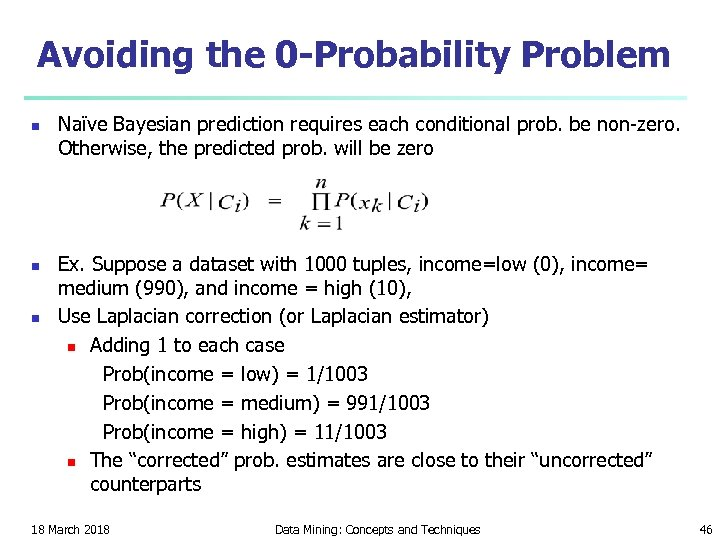Avoiding the 0 -Probability Problem n n n Naïve Bayesian prediction requires each conditional prob. be non-zero. Otherwise, the predicted prob. will be zero Ex. Suppose a dataset with 1000 tuples, income=low (0), income= medium (990), and income = high (10), Use Laplacian correction (or Laplacian estimator) n Adding 1 to each case Prob(income = low) = 1/1003 Prob(income = medium) = 991/1003 Prob(income = high) = 11/1003 n The “corrected” prob. estimates are close to their “uncorrected” counterparts 18 March 2018 Data Mining: Concepts and Techniques 46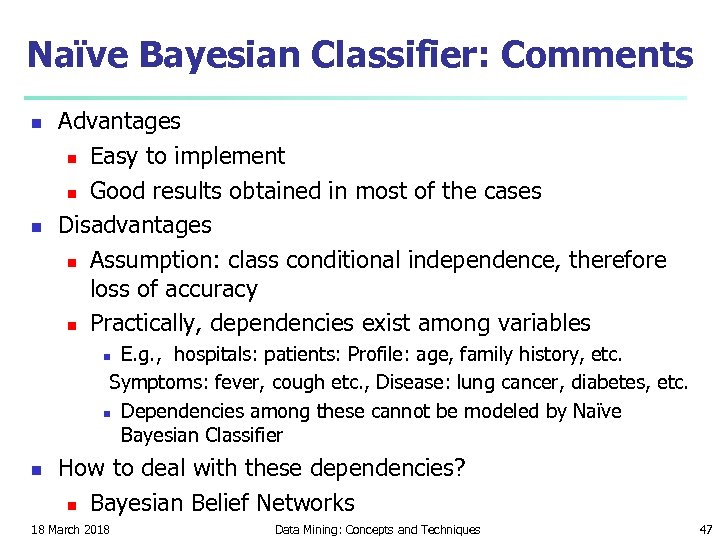Naïve Bayesian Classifier: Comments n n Advantages n Easy to implement n Good results obtained in most of the cases Disadvantages n Assumption: class conditional independence, therefore loss of accuracy n Practically, dependencies exist among variables E. g. , hospitals: patients: Profile: age, family history, etc. Symptoms: fever, cough etc. , Disease: lung cancer, diabetes, etc. n Dependencies among these cannot be modeled by Naïve Bayesian Classifier n n How to deal with these dependencies? n Bayesian Belief Networks 18 March 2018 Data Mining: Concepts and Techniques 47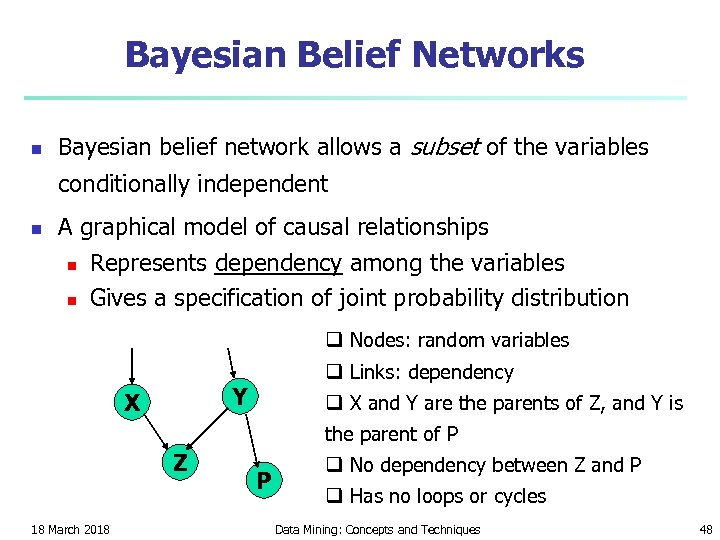Bayesian Belief Networks n Bayesian belief network allows a subset of the variables conditionally independent n A graphical model of causal relationships n n Represents dependency among the variables Gives a specification of joint probability distribution q Nodes: random variables q Links: dependency Y X q X and Y are the parents of Z, and Y is the parent of P Z 18 March 2018 P q No dependency between Z and P q Has no loops or cycles Data Mining: Concepts and Techniques 48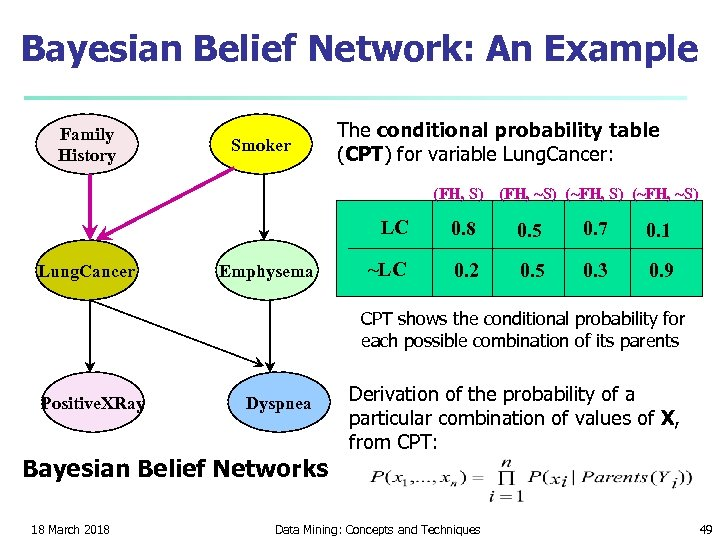Bayesian Belief Network: An Example Family History Smoker The conditional probability table (CPT) for variable Lung. Cancer: (FH, S) (FH, ~S) (~FH, ~S) LC Lung. Cancer Emphysema 0. 8 0. 5 0. 7 0. 1 ~LC 0. 2 0. 5 0. 3 0. 9 CPT shows the conditional probability for each possible combination of its parents Positive. XRay Dyspnea Bayesian Belief Networks 18 March 2018 Derivation of the probability of a particular combination of values of X, from CPT: Data Mining: Concepts and Techniques 49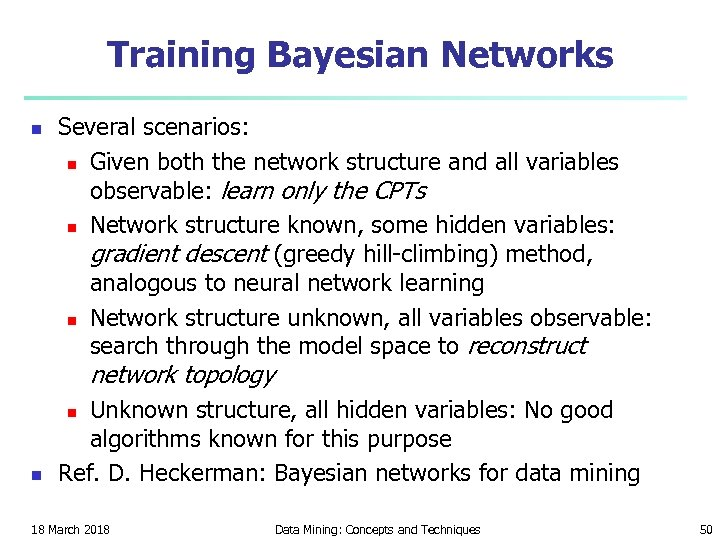Training Bayesian Networks n Several scenarios: n Given both the network structure and all variables observable: learn only the CPTs n Network structure known, some hidden variables: gradient descent (greedy hill-climbing) method, analogous to neural network learning n Network structure unknown, all variables observable: search through the model space to reconstruct network topology Unknown structure, all hidden variables: No good algorithms known for this purpose Ref. D. Heckerman: Bayesian networks for data mining n n 18 March 2018 Data Mining: Concepts and Techniques 50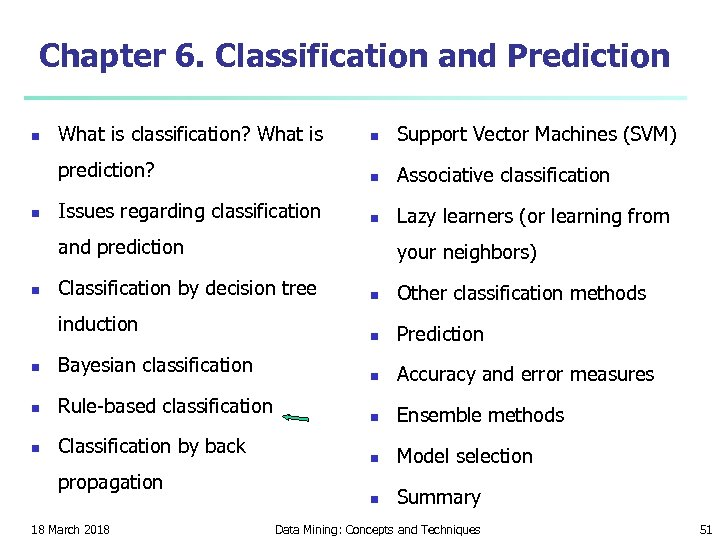Chapter 6. Classification and Prediction n What is classification? What is n Support Vector Machines (SVM) prediction? n n Associative classification Issues regarding classification n Lazy learners (or learning from and prediction n your neighbors) Classification by decision tree induction n Bayesian classification n Rule-based classification n Classification by back propagation 18 March 2018 n Other classification methods n Prediction n Accuracy and error measures n Ensemble methods n Model selection n Summary Data Mining: Concepts and Techniques 51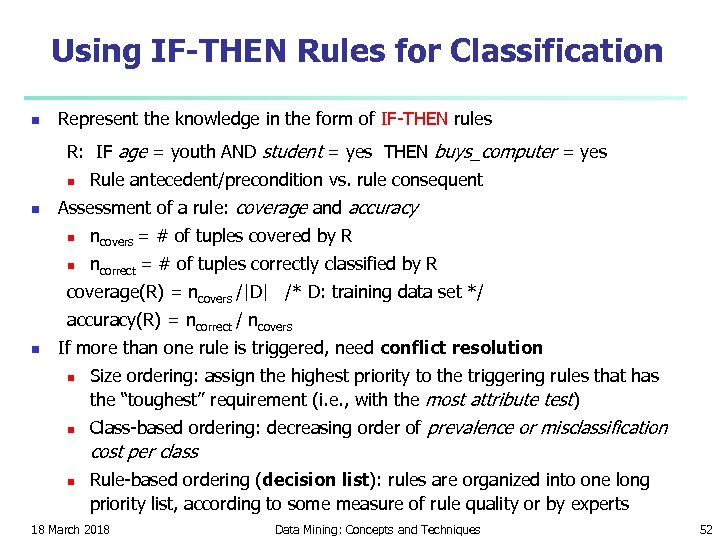Using IF-THEN Rules for Classification n Represent the knowledge in the form of IF-THEN rules R: IF age = youth AND student = yes THEN buys_computer = yes n n Rule antecedent/precondition vs. rule consequent Assessment of a rule: coverage and accuracy n ncovers = # of tuples covered by R n ncorrect = # of tuples correctly classified by R coverage(R) = ncovers /|D| /* D: training data set */ accuracy(R) = ncorrect / ncovers n If more than one rule is triggered, need conflict resolution n n Size ordering: assign the highest priority to the triggering rules that has the “toughest” requirement (i. e. , with the most attribute test) Class-based ordering: decreasing order of prevalence or misclassification cost per class n Rule-based ordering (decision list): rules are organized into one long priority list, according to some measure of rule quality or by experts 18 March 2018 Data Mining: Concepts and Techniques 52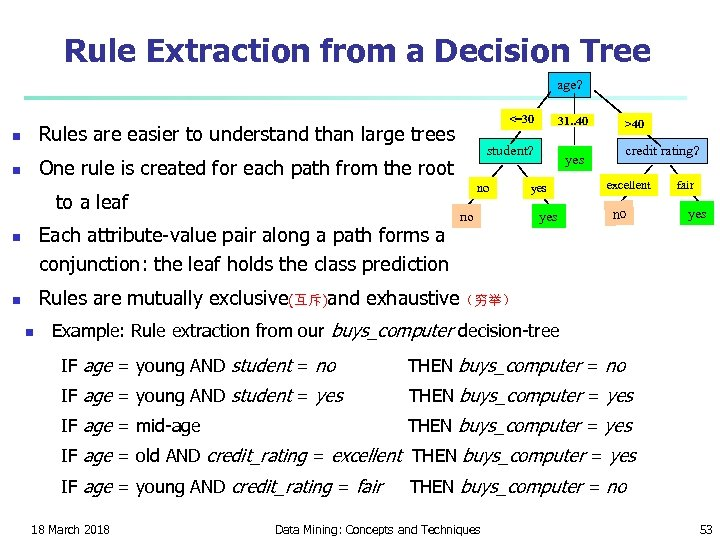Rule Extraction from a Decision Tree age? <=30 Rules are easier to understand than large trees n student? One rule is created for each path from the root n no to a leaf Each attribute-value pair along a path forms a conjunction: the leaf holds the class prediction n 31. . 40 no >40 credit rating? yes yes excellent no fair yes Rules are mutually exclusive(互斥)and exhaustive（穷举） n n Example: Rule extraction from our buys_computer decision-tree IF age = young AND student = no THEN buys_computer = no IF age = young AND student = yes THEN buys_computer = yes IF age = mid-age THEN buys_computer = yes IF age = old AND credit_rating = excellent THEN buys_computer = yes IF age = young AND credit_rating = fair 18 March 2018 THEN buys_computer = no Data Mining: Concepts and Techniques 53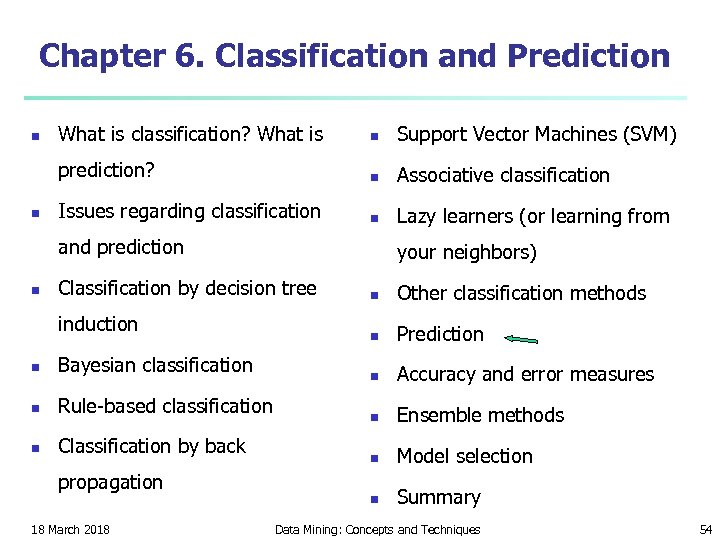Chapter 6. Classification and Prediction n What is classification? What is n Support Vector Machines (SVM) prediction? n n Associative classification Issues regarding classification n Lazy learners (or learning from and prediction n your neighbors) Classification by decision tree induction n Bayesian classification n Rule-based classification n Classification by back propagation 18 March 2018 n Other classification methods n Prediction n Accuracy and error measures n Ensemble methods n Model selection n Summary Data Mining: Concepts and Techniques 54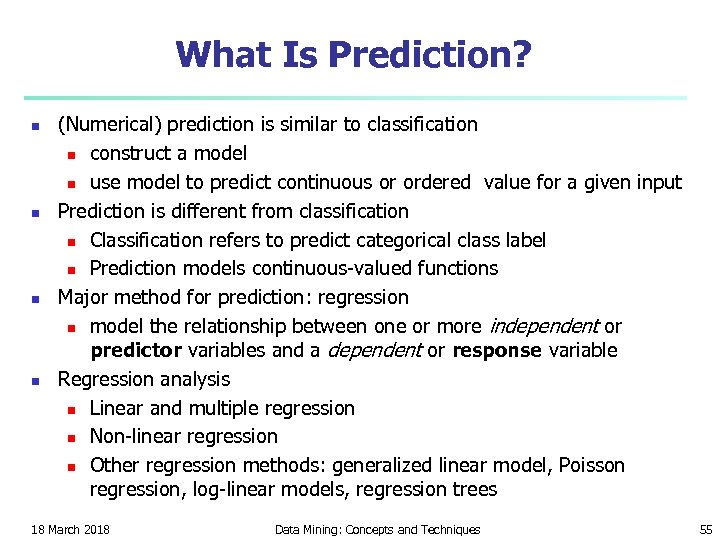What Is Prediction? n n (Numerical) prediction is similar to classification n construct a model n use model to predict continuous or ordered value for a given input Prediction is different from classification n Classification refers to predict categorical class label n Prediction models continuous-valued functions Major method for prediction: regression n model the relationship between one or more independent or predictor variables and a dependent or response variable Regression analysis n Linear and multiple regression n Non-linear regression n Other regression methods: generalized linear model, Poisson regression, log-linear models, regression trees 18 March 2018 Data Mining: Concepts and Techniques 55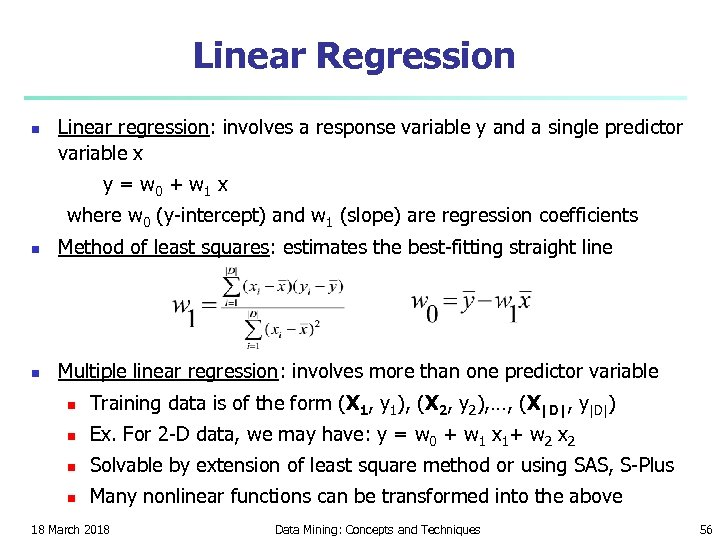Linear Regression n Linear regression: involves a response variable y and a single predictor variable x y = w 0 + w 1 x where w 0 (y-intercept) and w 1 (slope) are regression coefficients n Method of least squares: estimates the best-fitting straight line n Multiple linear regression: involves more than one predictor variable n Training data is of the form (X 1, y 1), (X 2, y 2), …, (X|D|, y|D|) n Ex. For 2 -D data, we may have: y = w 0 + w 1 x 1+ w 2 x 2 n Solvable by extension of least square method or using SAS, S-Plus n Many nonlinear functions can be transformed into the above 18 March 2018 Data Mining: Concepts and Techniques 56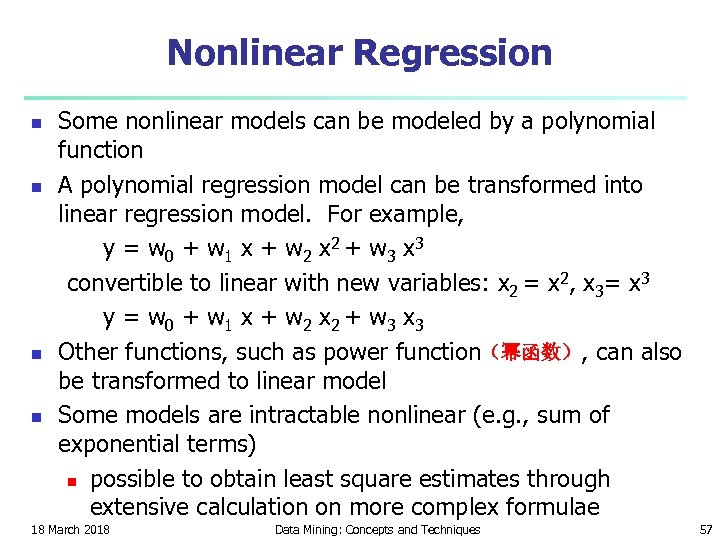Nonlinear Regression n n Some nonlinear models can be modeled by a polynomial function A polynomial regression model can be transformed into linear regression model. For example, y = w 0 + w 1 x + w 2 x 2 + w 3 x 3 convertible to linear with new variables: x 2 = x 2, x 3= x 3 y = w 0 + w 1 x + w 2 x 2 + w 3 x 3 Other functions, such as power function（幂函数）, can also be transformed to linear model Some models are intractable nonlinear (e. g. , sum of exponential terms) n possible to obtain least square estimates through extensive calculation on more complex formulae 18 March 2018 Data Mining: Concepts and Techniques 57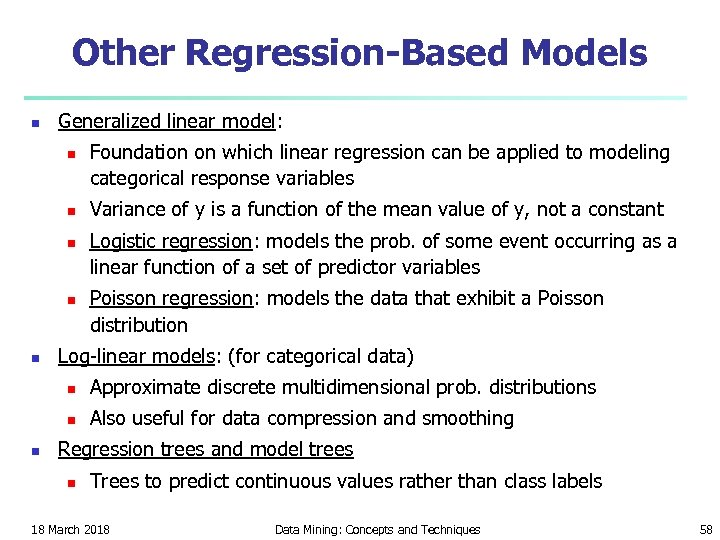Other Regression-Based Models n Generalized linear model: n n n Foundation on which linear regression can be applied to modeling categorical response variables Variance of y is a function of the mean value of y, not a constant Logistic regression: models the prob. of some event occurring as a linear function of a set of predictor variables Poisson regression: models the data that exhibit a Poisson distribution Log-linear models: (for categorical data) n n n Approximate discrete multidimensional prob. distributions Also useful for data compression and smoothing Regression trees and model trees n Trees to predict continuous values rather than class labels 18 March 2018 Data Mining: Concepts and Techniques 58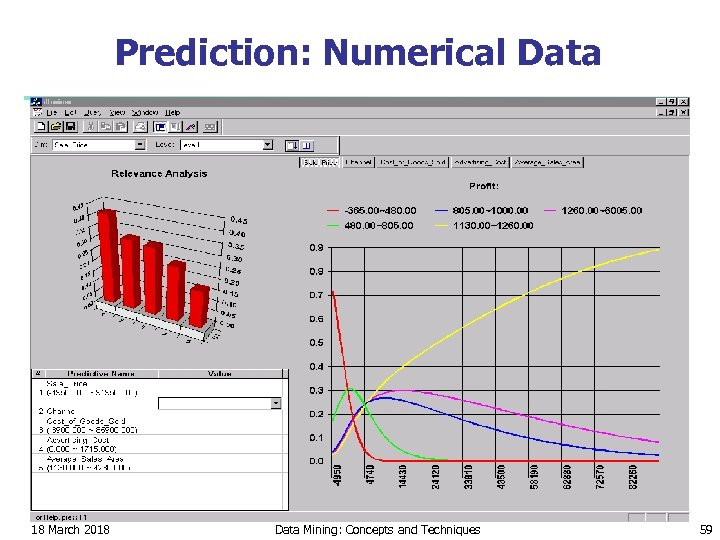Prediction: Numerical Data 18 March 2018 Data Mining: Concepts and Techniques 59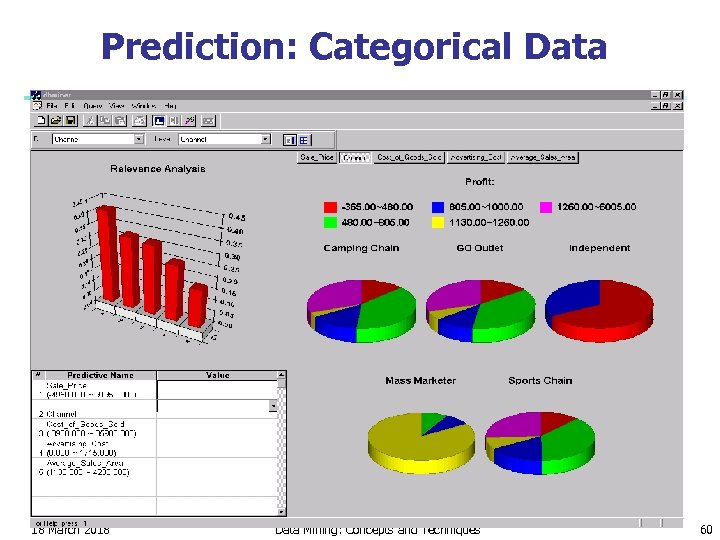Prediction: Categorical Data 18 March 2018 Data Mining: Concepts and Techniques 60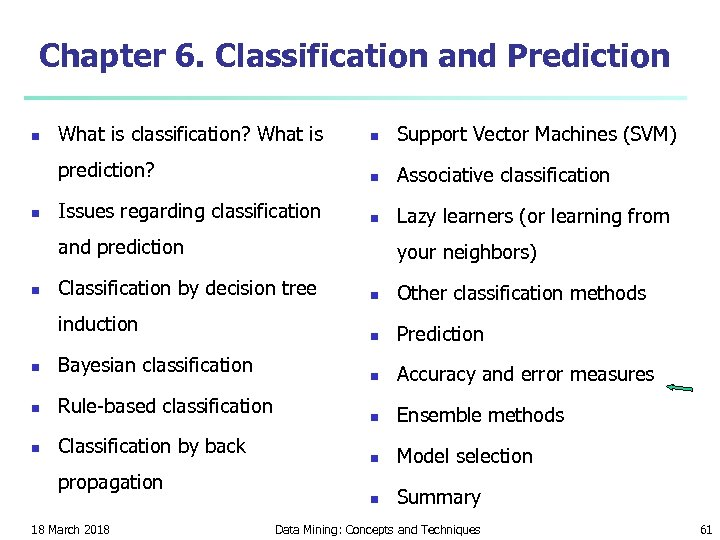Chapter 6. Classification and Prediction n What is classification? What is n Support Vector Machines (SVM) prediction? n n Associative classification Issues regarding classification n Lazy learners (or learning from and prediction n your neighbors) Classification by decision tree induction n Bayesian classification n Rule-based classification n Classification by back propagation 18 March 2018 n Other classification methods n Prediction n Accuracy and error measures n Ensemble methods n Model selection n Summary Data Mining: Concepts and Techniques 61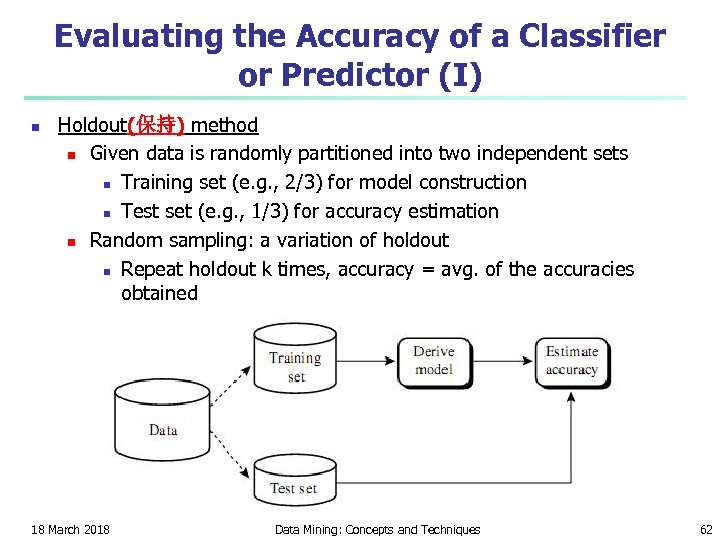Evaluating the Accuracy of a Classifier or Predictor (I) n Holdout(保持) method n Given data is randomly partitioned into two independent sets n Training set (e. g. , 2/3) for model construction n Test set (e. g. , 1/3) for accuracy estimation n Random sampling: a variation of holdout n Repeat holdout k times, accuracy = avg. of the accuracies obtained 18 March 2018 Data Mining: Concepts and Techniques 62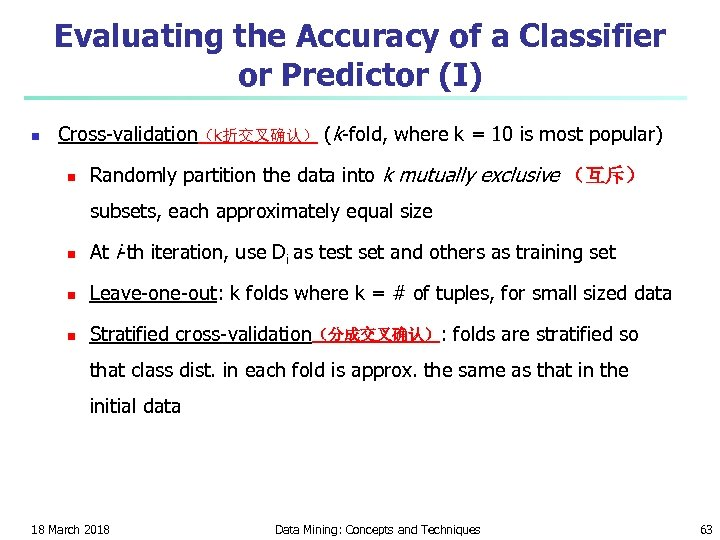Evaluating the Accuracy of a Classifier or Predictor (I) n Cross-validation（k折交叉确认） (k-fold, where k = 10 is most popular) n Randomly partition the data into k mutually exclusive （互斥） subsets, each approximately equal size n At i-th iteration, use Di as test set and others as training set n Leave-one-out: k folds where k = # of tuples, for small sized data n Stratified cross-validation（分成交叉确认）: folds are stratified so that class dist. in each fold is approx. the same as that in the initial data 18 March 2018 Data Mining: Concepts and Techniques 63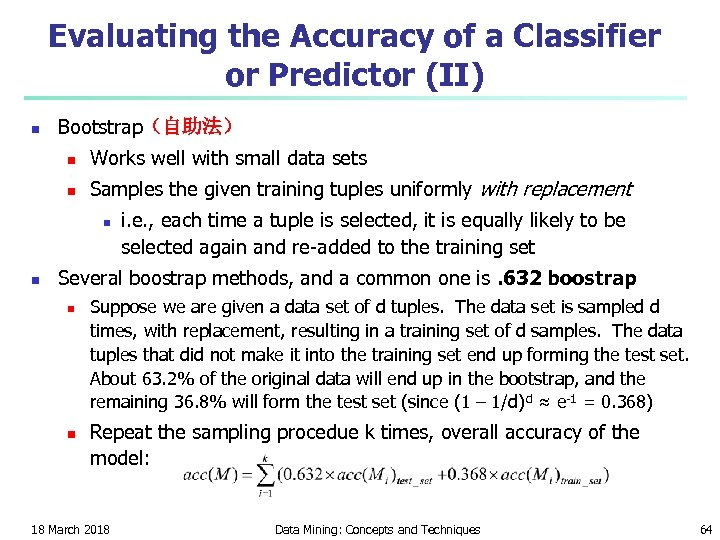Evaluating the Accuracy of a Classifier or Predictor (II) n Bootstrap（自助法） n Works well with small data sets n Samples the given training tuples uniformly with replacement n n i. e. , each time a tuple is selected, it is equally likely to be selected again and re-added to the training set Several boostrap methods, and a common one is. 632 boostrap n n Suppose we are given a data set of d tuples. The data set is sampled d times, with replacement, resulting in a training set of d samples. The data tuples that did not make it into the training set end up forming the test set. About 63. 2% of the original data will end up in the bootstrap, and the remaining 36. 8% will form the test set (since (1 – 1/d) d ≈ e-1 = 0. 368) Repeat the sampling procedue k times, overall accuracy of the model: 18 March 2018 Data Mining: Concepts and Techniques 64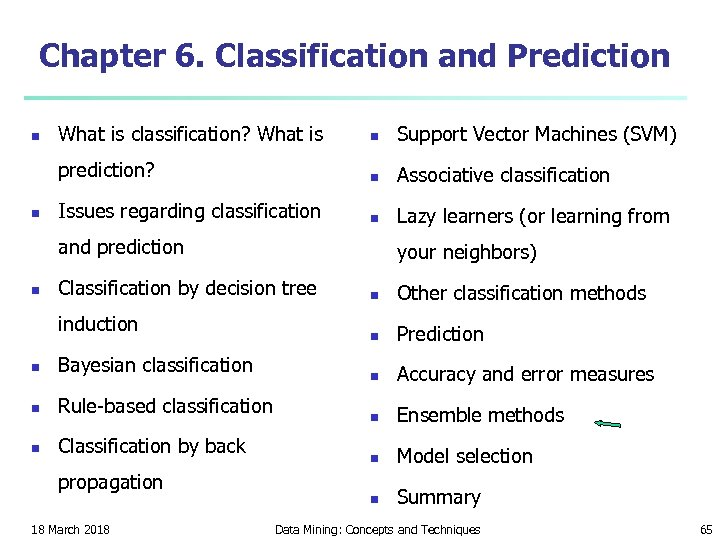Chapter 6. Classification and Prediction n What is classification? What is n Support Vector Machines (SVM) prediction? n n Associative classification Issues regarding classification n Lazy learners (or learning from and prediction n your neighbors) Classification by decision tree induction n Bayesian classification n Rule-based classification n Classification by back propagation 18 March 2018 n Other classification methods n Prediction n Accuracy and error measures n Ensemble methods n Model selection n Summary Data Mining: Concepts and Techniques 65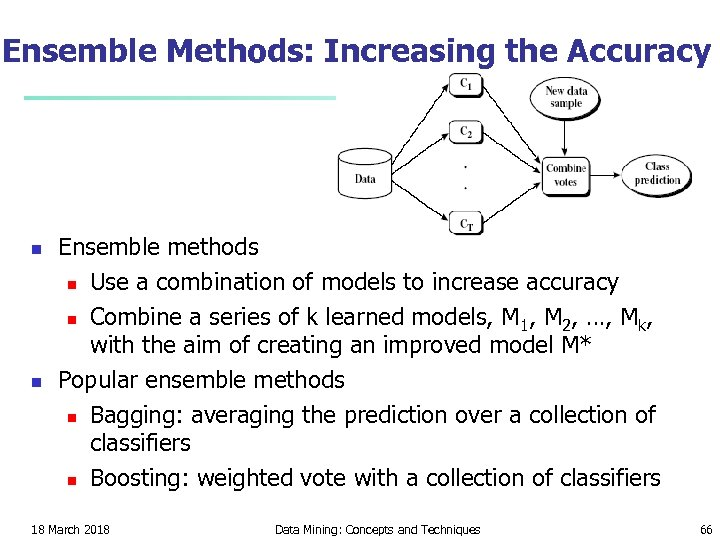Ensemble Methods: Increasing the Accuracy n n Ensemble methods n Use a combination of models to increase accuracy n Combine a series of k learned models, M 1, M 2, …, Mk, with the aim of creating an improved model M* Popular ensemble methods n Bagging: averaging the prediction over a collection of classifiers n Boosting: weighted vote with a collection of classifiers 18 March 2018 Data Mining: Concepts and Techniques 66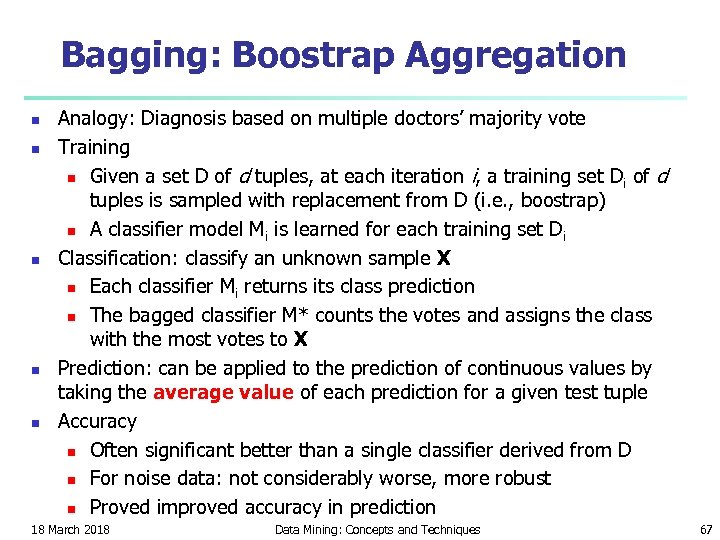Bagging: Boostrap Aggregation n n Analogy: Diagnosis based on multiple doctors’ majority vote Training n Given a set D of d tuples, at each iteration i, a training set Di of d tuples is sampled with replacement from D (i. e. , boostrap) n A classifier model Mi is learned for each training set D i Classification: classify an unknown sample X n Each classifier Mi returns its class prediction n The bagged classifier M* counts the votes and assigns the class with the most votes to X Prediction: can be applied to the prediction of continuous values by taking the average value of each prediction for a given test tuple Accuracy n Often significant better than a single classifier derived from D n For noise data: not considerably worse, more robust n Proved improved accuracy in prediction 18 March 2018 Data Mining: Concepts and Techniques 67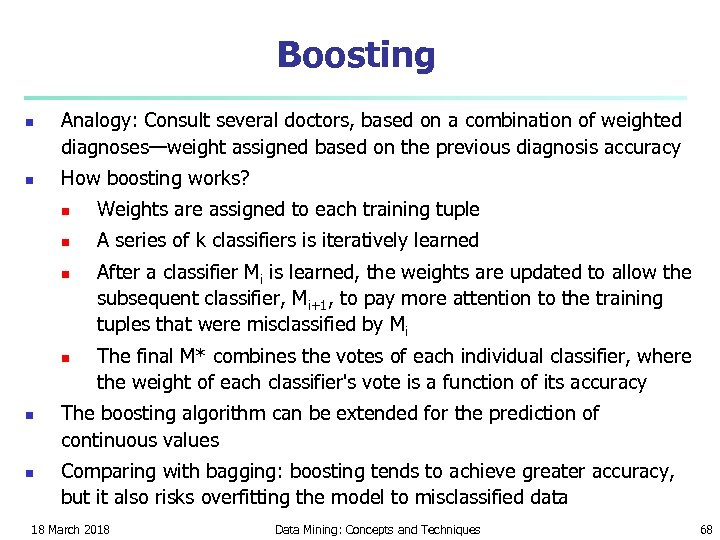Boosting n n Analogy: Consult several doctors, based on a combination of weighted diagnoses—weight assigned based on the previous diagnosis accuracy How boosting works? n Weights are assigned to each training tuple n A series of k classifiers is iteratively learned n n After a classifier Mi is learned, the weights are updated to allow the subsequent classifier, Mi+1, to pay more attention to the training tuples that were misclassified by Mi The final M* combines the votes of each individual classifier, where the weight of each classifier's vote is a function of its accuracy The boosting algorithm can be extended for the prediction of continuous values Comparing with bagging: boosting tends to achieve greater accuracy, but it also risks overfitting the model to misclassified data 18 March 2018 Data Mining: Concepts and Techniques 68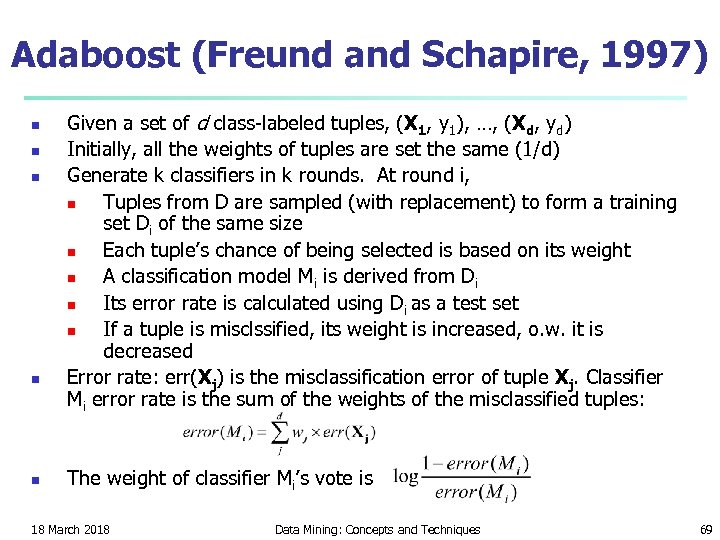Adaboost (Freund and Schapire, 1997) n n n Given a set of d class-labeled tuples, (X 1, y 1), …, (Xd, yd) Initially, all the weights of tuples are set the same (1/d) Generate k classifiers in k rounds. At round i, n Tuples from D are sampled (with replacement) to form a training set Di of the same size n Each tuple’s chance of being selected is based on its weight n A classification model Mi is derived from Di n Its error rate is calculated using Di as a test set n If a tuple is misclssified, its weight is increased, o. w. it is decreased Error rate: err(Xj) is the misclassification error of tuple Xj. Classifier Mi error rate is the sum of the weights of the misclassified tuples: The weight of classifier Mi’s vote is 18 March 2018 Data Mining: Concepts and Techniques 69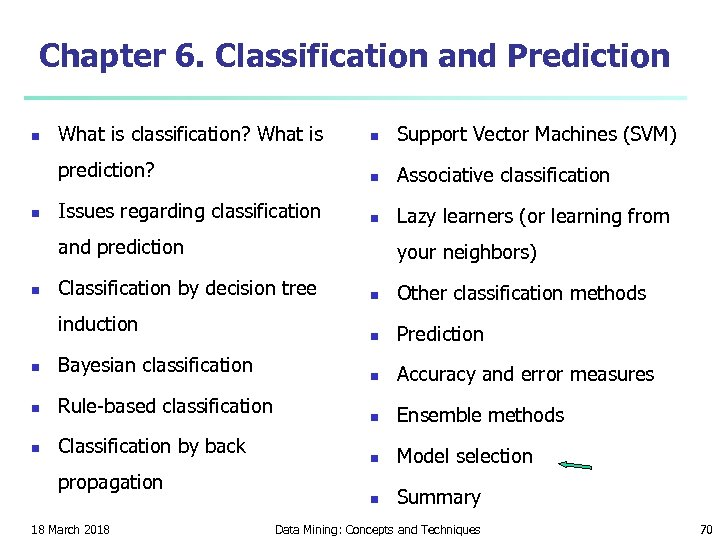Chapter 6. Classification and Prediction n What is classification? What is n Support Vector Machines (SVM) prediction? n n Associative classification Issues regarding classification n Lazy learners (or learning from and prediction n your neighbors) Classification by decision tree induction n Bayesian classification n Rule-based classification n Classification by back propagation 18 March 2018 n Other classification methods n Prediction n Accuracy and error measures n Ensemble methods n Model selection n Summary Data Mining: Concepts and Techniques 70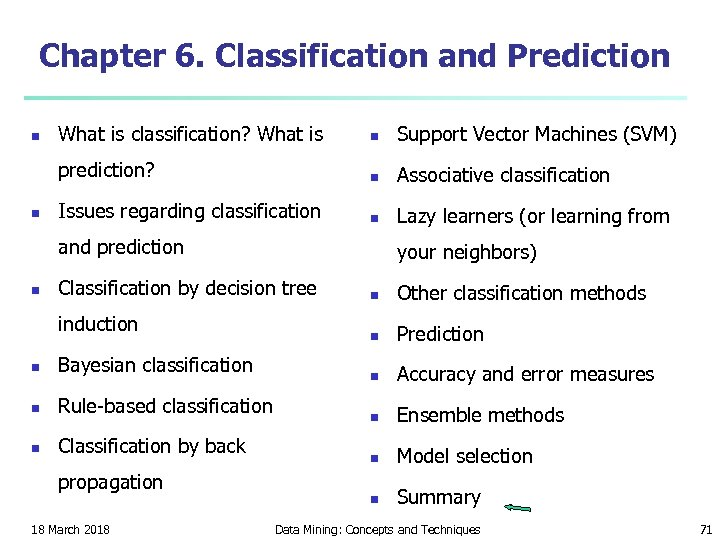Chapter 6. Classification and Prediction n What is classification? What is n Support Vector Machines (SVM) prediction? n n Associative classification Issues regarding classification n Lazy learners (or learning from and prediction n your neighbors) Classification by decision tree induction n Bayesian classification n Rule-based classification n Classification by back propagation 18 March 2018 n Other classification methods n Prediction n Accuracy and error measures n Ensemble methods n Model selection n Summary Data Mining: Concepts and Techniques 71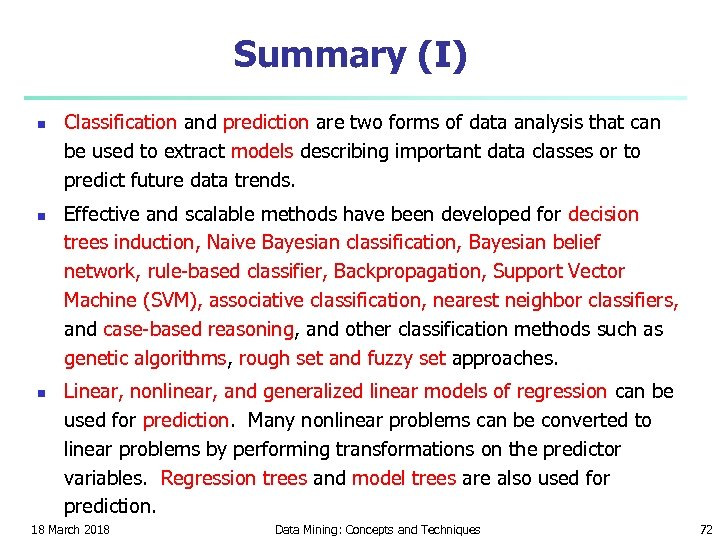Summary (I) n n n Classification and prediction are two forms of data analysis that can be used to extract models describing important data classes or to predict future data trends. Effective and scalable methods have been developed for decision trees induction, Naive Bayesian classification, Bayesian belief network, rule-based classifier, Backpropagation, Support Vector Machine (SVM), associative classification, nearest neighbor classifiers, and case-based reasoning, and other classification methods such as genetic algorithms, rough set and fuzzy set approaches. Linear, nonlinear, and generalized linear models of regression can be used for prediction. Many nonlinear problems can be converted to linear problems by performing transformations on the predictor variables. Regression trees and model trees are also used for prediction. 18 March 2018 Data Mining: Concepts and Techniques 72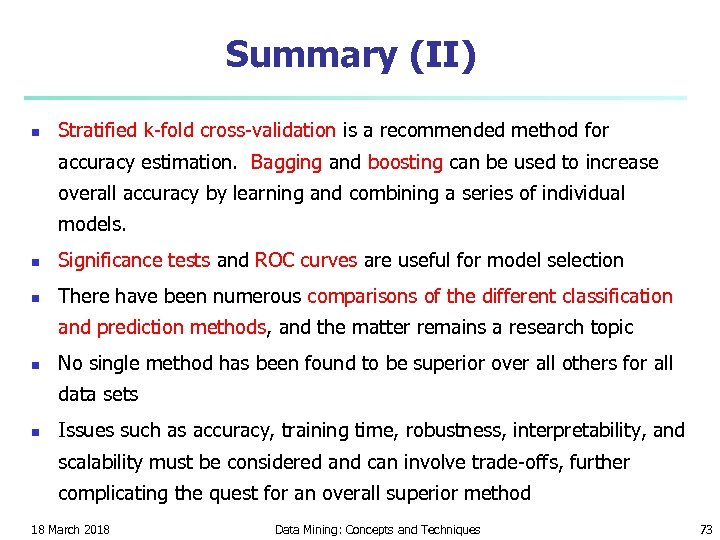Summary (II) n Stratified k-fold cross-validation is a recommended method for accuracy estimation. Bagging and boosting can be used to increase overall accuracy by learning and combining a series of individual models. n Significance tests and ROC curves are useful for model selection n There have been numerous comparisons of the different classification and prediction methods, and the matter remains a research topic n No single method has been found to be superior over all others for all data sets n Issues such as accuracy, training time, robustness, interpretability, and scalability must be considered and can involve trade-offs, further complicating the quest for an overall superior method 18 March 2018 Data Mining: Concepts and Techniques 73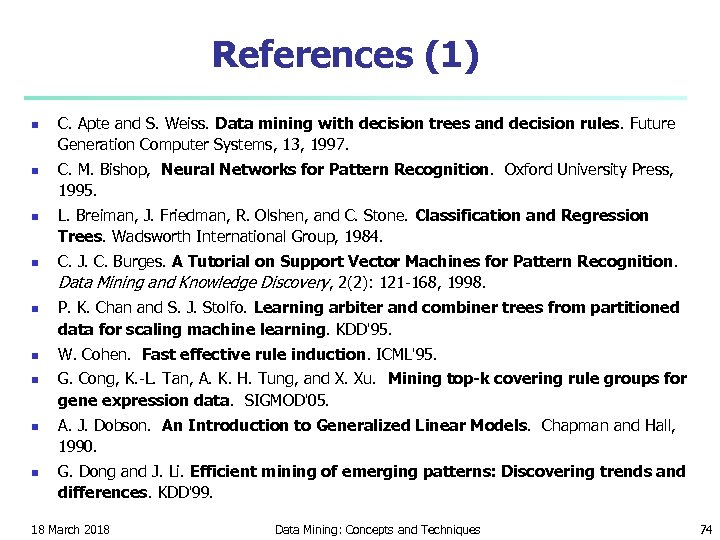References (1) n n n n n C. Apte and S. Weiss. Data mining with decision trees and decision rules. Future Generation Computer Systems, 13, 1997. C. M. Bishop, Neural Networks for Pattern Recognition. Oxford University Press, 1995. L. Breiman, J. Friedman, R. Olshen, and C. Stone. Classification and Regression Trees. Wadsworth International Group, 1984. C. J. C. Burges. A Tutorial on Support Vector Machines for Pattern Recognition. Data Mining and Knowledge Discovery, 2(2): 121 -168, 1998. P. K. Chan and S. J. Stolfo. Learning arbiter and combiner trees from partitioned data for scaling machine learning. KDD'95. W. Cohen. Fast effective rule induction. ICML'95. G. Cong, K. -L. Tan, A. K. H. Tung, and X. Xu. Mining top-k covering rule groups for gene expression data. SIGMOD'05. A. J. Dobson. An Introduction to Generalized Linear Models. Chapman and Hall, 1990. G. Dong and J. Li. Efficient mining of emerging patterns: Discovering trends and differences. KDD'99. 18 March 2018 Data Mining: Concepts and Techniques 74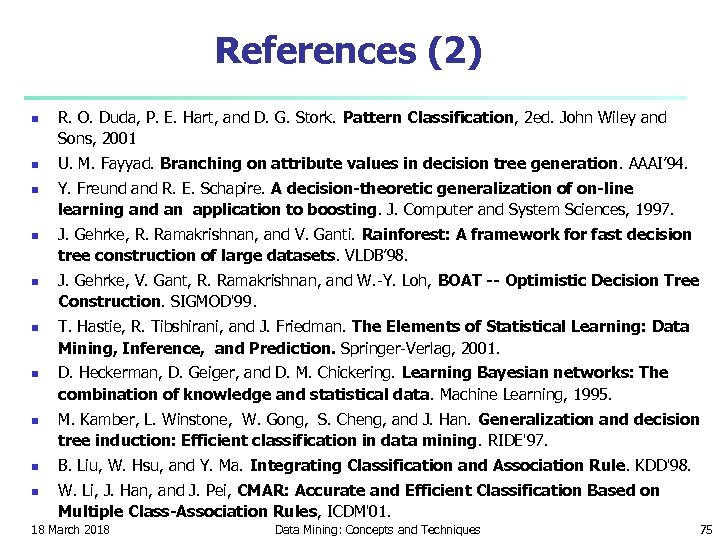References (2) n n n n n R. O. Duda, P. E. Hart, and D. G. Stork. Pattern Classification, 2 ed. John Wiley and Sons, 2001 U. M. Fayyad. Branching on attribute values in decision tree generation. AAAI’ 94. Y. Freund and R. E. Schapire. A decision-theoretic generalization of on-line learning and an application to boosting. J. Computer and System Sciences, 1997. J. Gehrke, R. Ramakrishnan, and V. Ganti. Rainforest: A framework for fast decision tree construction of large datasets. VLDB’ 98. J. Gehrke, V. Gant, R. Ramakrishnan, and W. -Y. Loh, BOAT -- Optimistic Decision Tree Construction. SIGMOD'99. T. Hastie, R. Tibshirani, and J. Friedman. The Elements of Statistical Learning: Data Mining, Inference, and Prediction. Springer-Verlag, 2001. D. Heckerman, D. Geiger, and D. M. Chickering. Learning Bayesian networks: The combination of knowledge and statistical data. Machine Learning, 1995. M. Kamber, L. Winstone, W. Gong, S. Cheng, and J. Han. Generalization and decision tree induction: Efficient classification in data mining. RIDE'97. B. Liu, W. Hsu, and Y. Ma. Integrating Classification and Association Rule. KDD'98. W. Li, J. Han, and J. Pei, CMAR: Accurate and Efficient Classification Based on Multiple Class-Association Rules, ICDM'01. 18 March 2018 Data Mining: Concepts and Techniques 75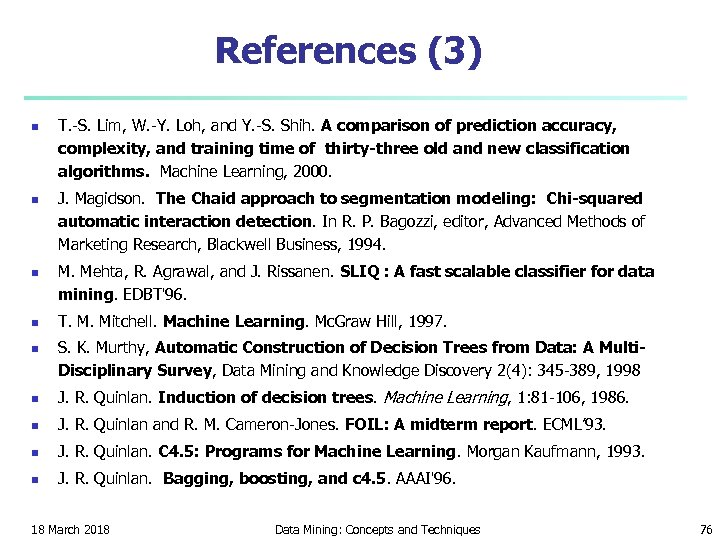References (3) n n n T. -S. Lim, W. -Y. Loh, and Y. -S. Shih. A comparison of prediction accuracy, complexity, and training time of thirty-three old and new classification algorithms. Machine Learning, 2000. J. Magidson. The Chaid approach to segmentation modeling: Chi-squared automatic interaction detection. In R. P. Bagozzi, editor, Advanced Methods of Marketing Research, Blackwell Business, 1994. M. Mehta, R. Agrawal, and J. Rissanen. SLIQ : A fast scalable classifier for data mining. EDBT'96. T. M. Mitchell. Machine Learning. Mc. Graw Hill, 1997. S. K. Murthy, Automatic Construction of Decision Trees from Data: A Multi. Disciplinary Survey, Data Mining and Knowledge Discovery 2(4): 345 -389, 1998 n J. R. Quinlan. Induction of decision trees. Machine Learning, 1: 81 -106, 1986. n J. R. Quinlan and R. M. Cameron-Jones. FOIL: A midterm report. ECML’ 93. n J. R. Quinlan. C 4. 5: Programs for Machine Learning. Morgan Kaufmann, 1993. n J. R. Quinlan. Bagging, boosting, and c 4. 5. AAAI'96. 18 March 2018 Data Mining: Concepts and Techniques 76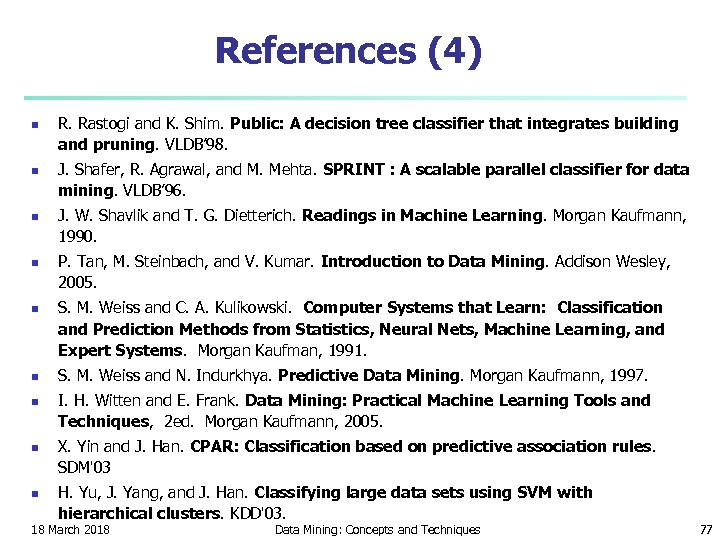References (4) n n n n n R. Rastogi and K. Shim. Public: A decision tree classifier that integrates building and pruning. VLDB’ 98. J. Shafer, R. Agrawal, and M. Mehta. SPRINT : A scalable parallel classifier for data mining. VLDB’ 96. J. W. Shavlik and T. G. Dietterich. Readings in Machine Learning. Morgan Kaufmann, 1990. P. Tan, M. Steinbach, and V. Kumar. Introduction to Data Mining. Addison Wesley, 2005. S. M. Weiss and C. A. Kulikowski. Computer Systems that Learn: Classification and Prediction Methods from Statistics, Neural Nets, Machine Learning, and Expert Systems. Morgan Kaufman, 1991. S. M. Weiss and N. Indurkhya. Predictive Data Mining. Morgan Kaufmann, 1997. I. H. Witten and E. Frank. Data Mining: Practical Machine Learning Tools and Techniques, 2 ed. Morgan Kaufmann, 2005. X. Yin and J. Han. CPAR: Classification based on predictive association rules. SDM'03 H. Yu, J. Yang, and J. Han. Classifying large data sets using SVM with hierarchical clusters. KDD'03. 18 March 2018 Data Mining: Concepts and Techniques 7718 March 2018 Data Mining: Concepts and Techniques 78